Publicité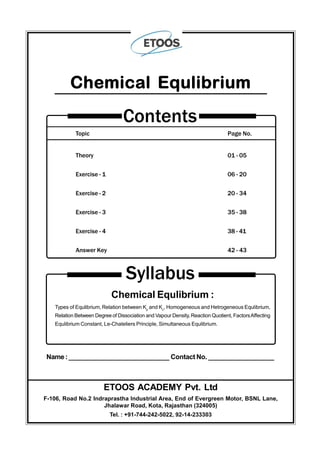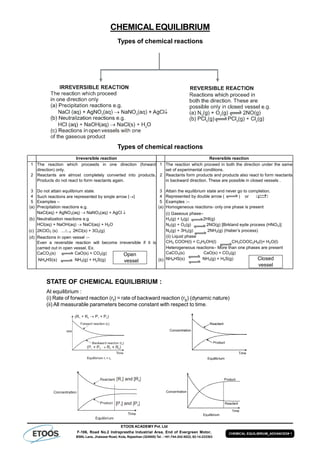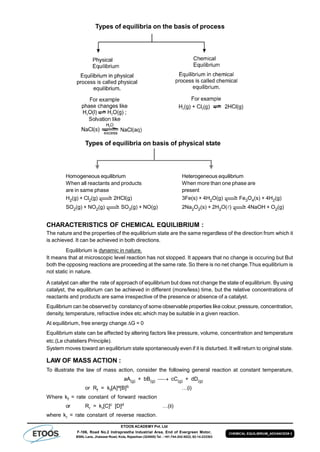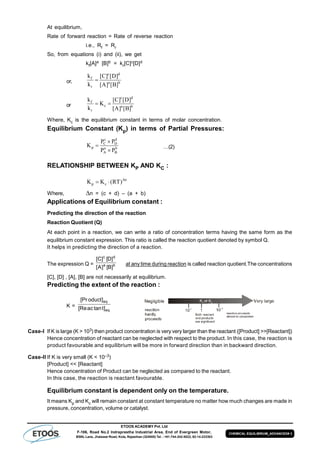Publicité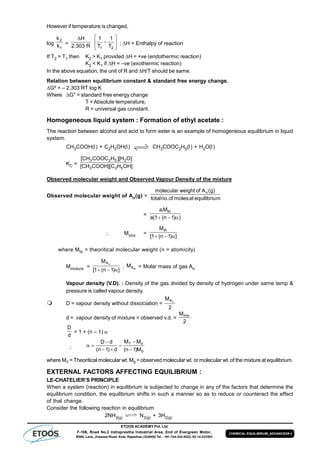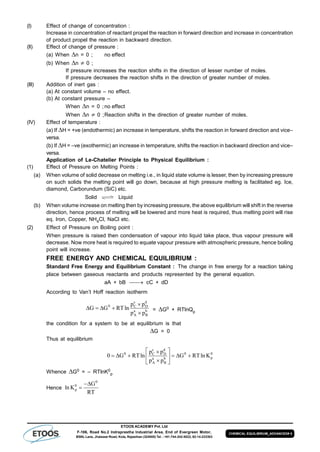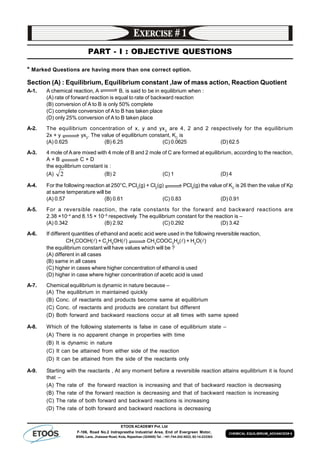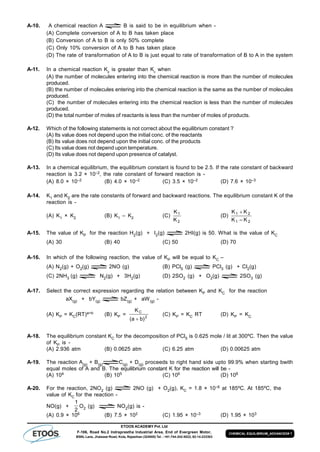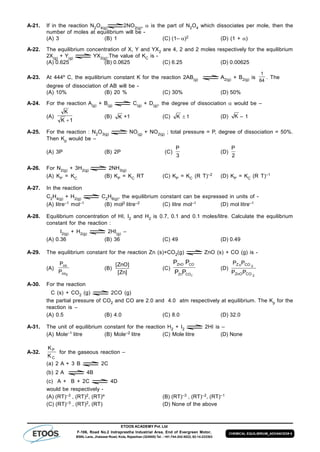Publicité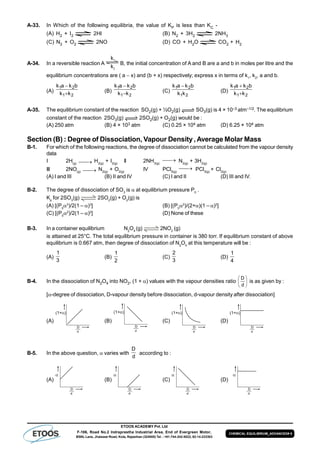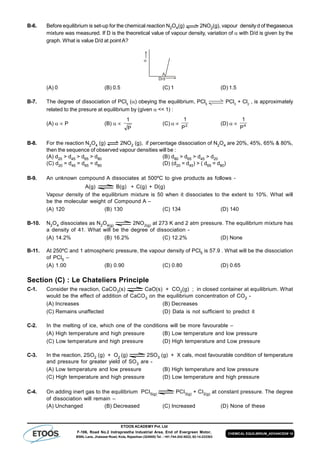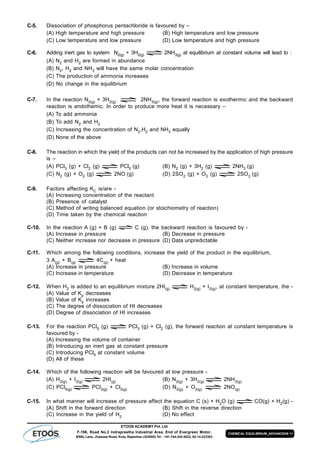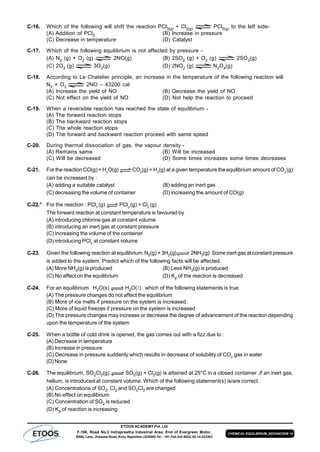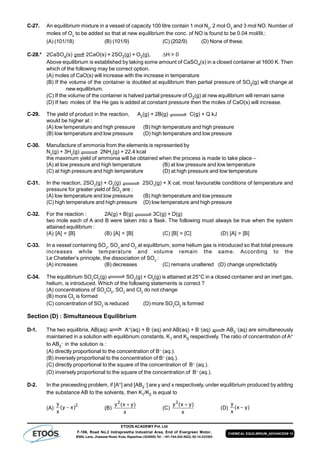Publicité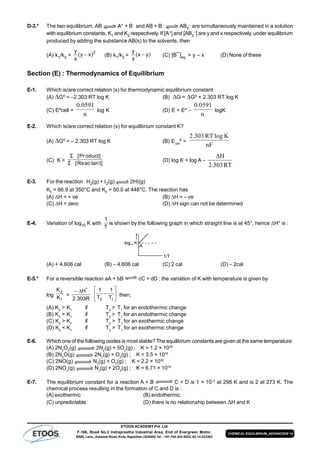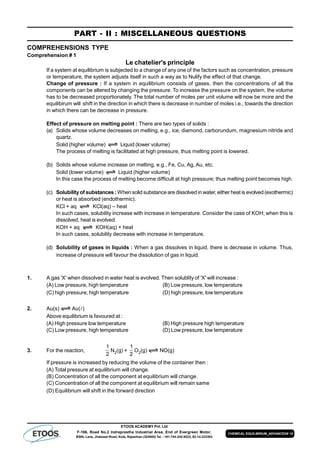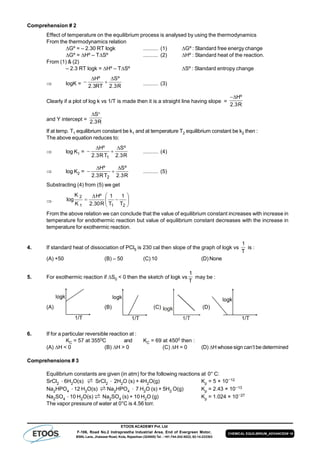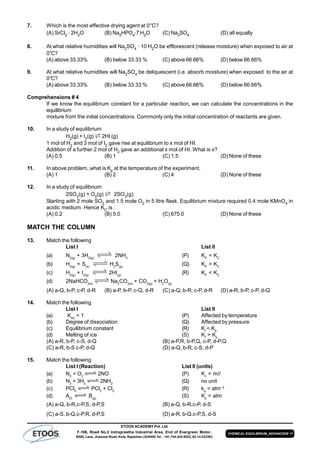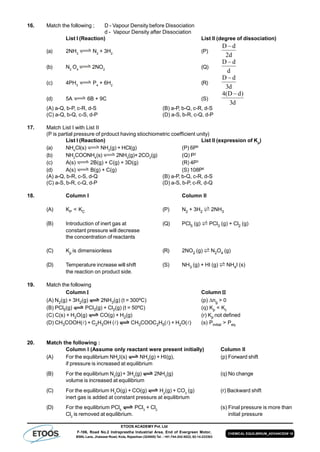Publicité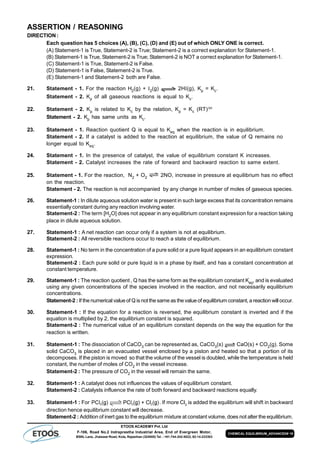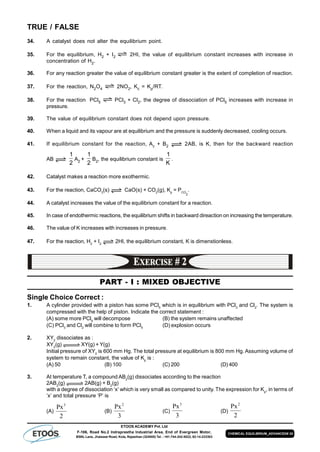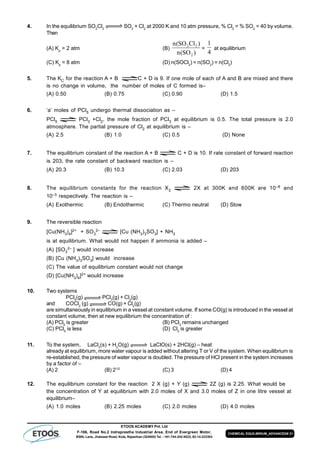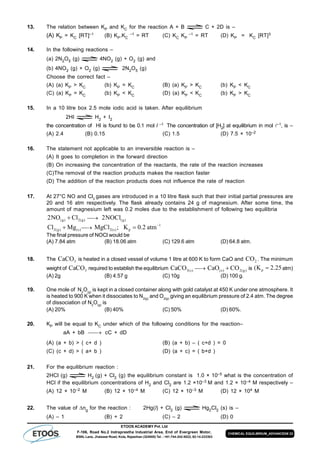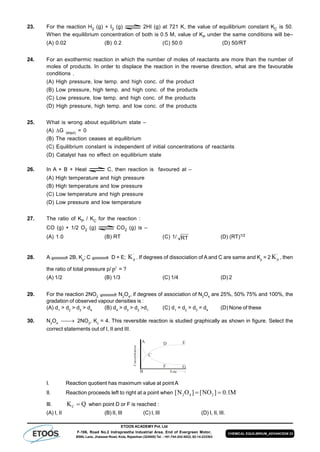Publicité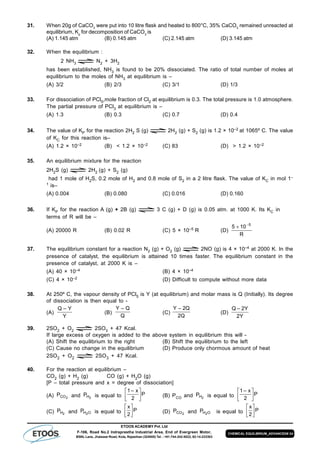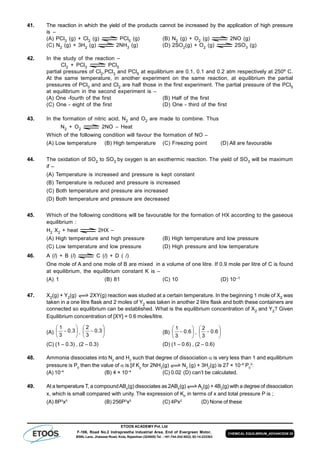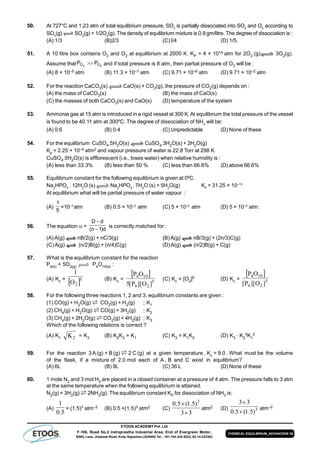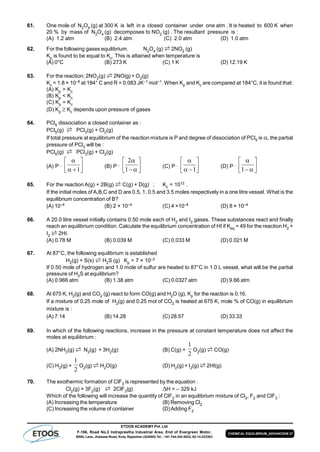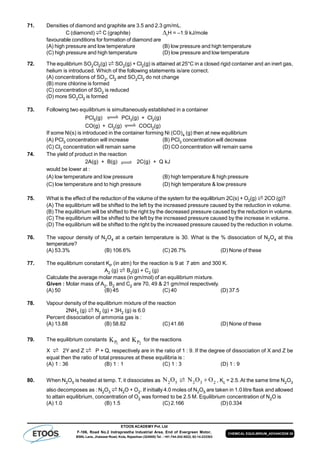Publicité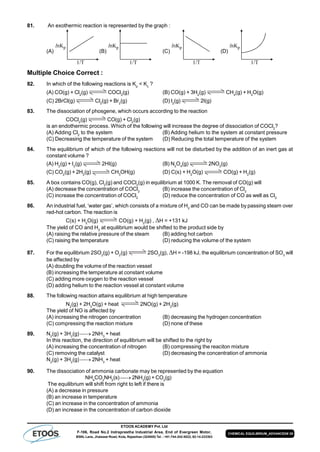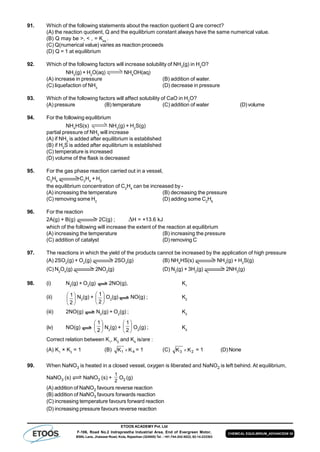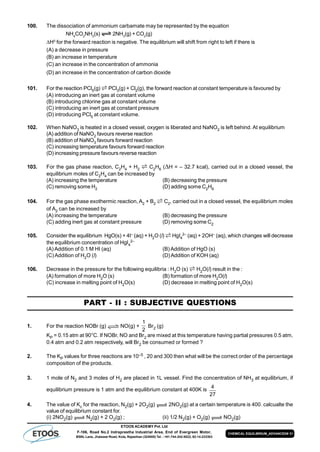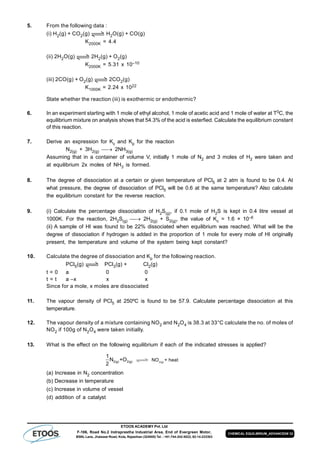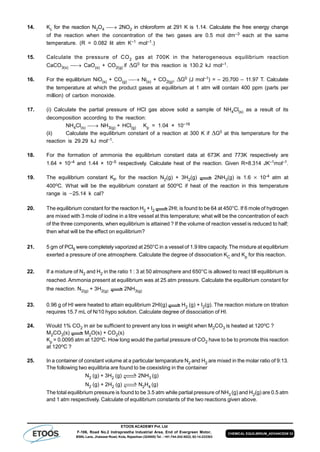Publicité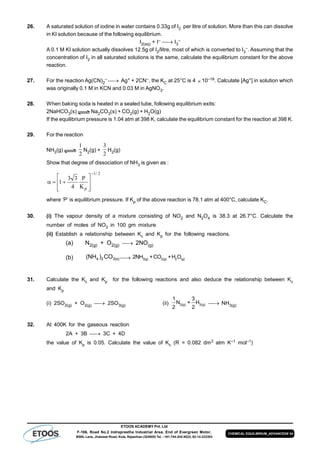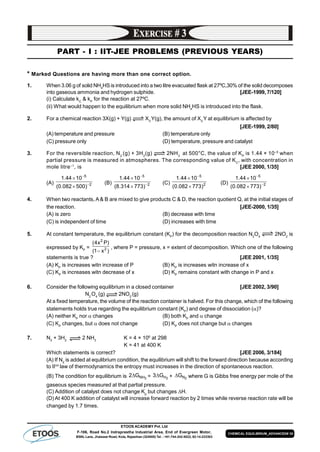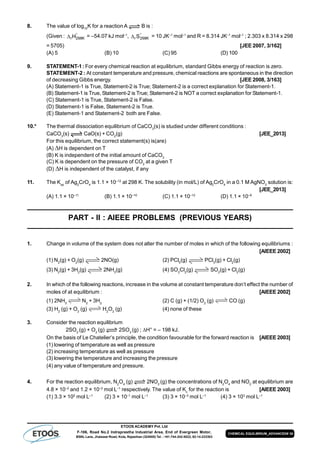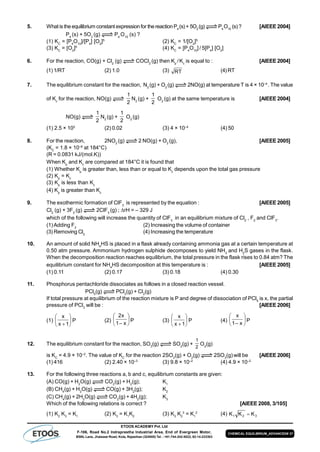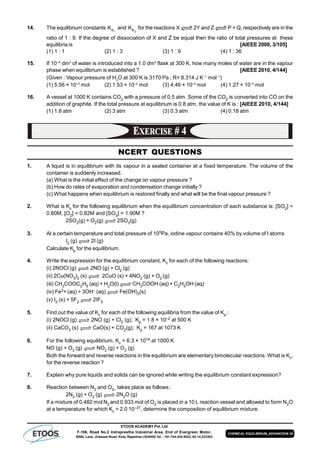Publicité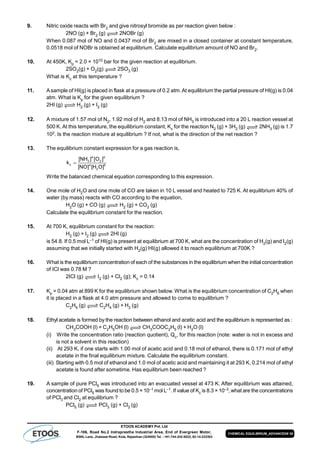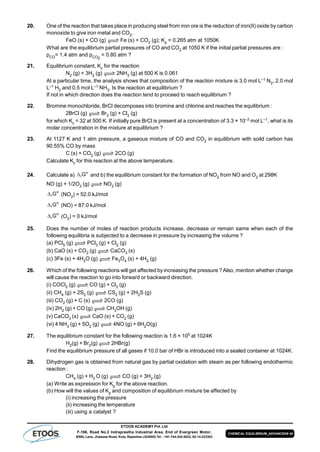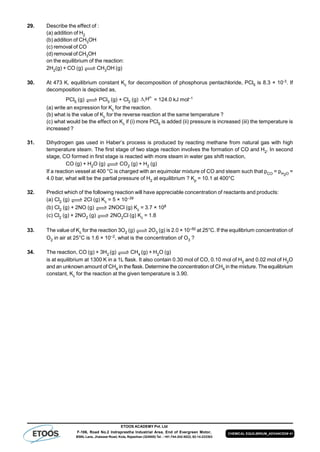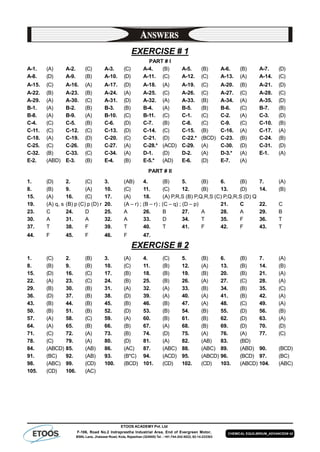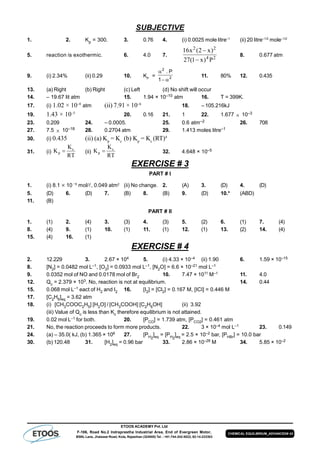Prochain SlideShareSynthetic-Composite Varieties & Hardw-w. law.pdf
Chargement dans ... 3
1 sur 44
Publicité

### Assignment chemical equilibrium_jh_sir-4168

1. Topic Page No. Theory 01 - 05 Exercise - 1 06 - 20 Exercise - 2 20 - 34 Exercise - 3 35 - 38 Exercise - 4 38 - 41 Answer Key 42 - 43 Contents Chemical Equlibrium Syllabus Chemical Equlibrium : Types of Equlibrium, Relation between Kp and Kc , Homogeneous and Hetrogeneous Equlibrium, Relation Between Degree of Dissociation and Vapour Density, Reaction Quotient, FactorsAffecting Equlibrium Constant, Le-Chateliers Principle, Simultaneous Equlibrium. Name:____________________________ Contact No. __________________ ETOOS ACADEMY Pvt. Ltd F-106, Road No.2 Indraprastha Industrial Area, End of Evergreen Motor, BSNL Lane, Jhalawar Road, Kota, Rajasthan (324005) Tel. : +91-744-242-5022, 92-14-233303
2. ETOOS ACADEMY Pvt. Ltd F-106, Road No.2 Indraprastha Industrial Area, End of Evergreen Motor, BSNL Lane, Jhalawar Road, Kota, Rajasthan (324005) Tel. : +91-744-242-5022, 92-14-233303 CHEMICAL EQUILIBRIUM_ADVANCED# 1 CHEMICAL EQUILIBRIUM Types of chemical reactions Types of chemical reactions Irreversible reaction Reversible reaction 1 The reaction which proceeds in one direction (forward direction) only. 1 The reaction which proceed in both the direction under the same set of experimental conditions. 2 Reactants are almost completely converted into products. Products do not react to form reactants again. 2 Reactants form products and products also react to form reactants in backward direction. These are possible in closed vessels . 3 Do not attain equilibrium state. 3 Attain the equilibrium state and never go to completion. 4 Such reactions are represented by single arrow {} 4 Represented by double arrow ( ) or 5 Examples – 5 Examples :– (a) Precipitation reactions e.g. NaCl(aq) + AgNO3(aq)  NaNO3(aq) + AgCl  (a) Homogeneous reactions- only one phase is present (b) Neutralization reactions e.g HCl(aq) + NaOH(aq)  NaCl(aq) + H2O (c) 2KClO3 (s) 2KCl(s) + 3O2(g) (d) Reactions in open vessel :– Even a reversible reaction will become irreversible if it is carried out in open vessel. Ex. CaCO3(s) CaO(s) + CO2(g) NH4HS(s) NH3(g) + H2S(g) (b)   ( ) (i) Gaseous phase– H2(g) + I2(g) 2HI(g) N2(g) + O2(g) 2NO(g) [Birkland eyde process (HNO3)] N2(g) + 3H2(g) 2NH3(g) (Haber’s process) (ii) Liquid phase CH3 COOH(l) + C2H5OH(l) CH3COOC2H5(l)+ H2O(l) Heterogeneous reactions– More than one phases are present CaCO3(s) CaO(s) + CO2(g) NH4HS(s) NH3(g) + H2S(g) Closed vessel Open vessel STATE OF CHEMICAL EQUILIBRIUM : At equilibrium : (i) Rate of forward reaction (rf) = rate of backward reaction (rb) (dynamic nature) (ii) All measurable parameters become constant with respect to time.
3. ETOOS ACADEMY Pvt. Ltd F-106, Road No.2 Indraprastha Industrial Area, End of Evergreen Motor, BSNL Lane, Jhalawar Road, Kota, Rajasthan (324005) Tel. : +91-744-242-5022, 92-14-233303 CHEMICAL EQUILIBRIUM_ADVANCED# 2 Types of equilibria on the basis of process Types of equilibria on basis of physical state  Homogeneous equilibrium Heterogeneous equilibrium When all reactants and products When more than one phase are are in same phase present H2(g) + Cl2(g) 2HCl(g) 3Fe(s) + 4H2O(g) Fe3O4(s) + 4H2(g) SO2(g) + NO2(g) SO3(g) + NO(g) 2Na2O2(s) + 2H2O() 4NaOH + O2(g) CHARACTERISTICS OF CHEMICAL EQUILIBRIUM : The nature and the properties of the equilibrium state are the same regardless of the direction from which it is achieved. It can be achieved in both directions. Equilibrium is dynamic in nature. It means that at microscopic level reaction has not stopped. It appears that no change is occuring but But both the opposing reactions are proceeding at the same rate. So there is no net change.Thus equilibrium is not static in nature. A catalyst can alter the rate of approach of equilibrium but does not change the state of equilibrium. By using catalyst, the equilibrium can be achieved in different (more/less) time, but the relative concentrations of reactants and products are same irrespective of the presence or absence of a catalyst. Equilibrium can be observed by constancy of some observable properties like colour, pressure, concentration, density, temperature, refractive index etc.which may be suitable in a given reaction. At equilibrium, free energy changeG = 0 Equilibrium state can be affected by altering factors like pressure, volume, concentration and temperature etc.(Le chateliers Principle). System moves toward an equilibrium state spontaneously even if it is disturbed. It will return to original state. LAW OF MASS ACTION : To illustrate the law of mass action, consider the following general reaction at constant temperature, aA(g) + bB(g)  cC(g) + dD(g) or Rf = kf[A]a[B]b …(i) Where kf = rate constant of forward reaction or Rr = kr[C]c [D]d …(ii) where kr = rate constant of reverse reaction.
4. ETOOS ACADEMY Pvt. Ltd F-106, Road No.2 Indraprastha Industrial Area, End of Evergreen Motor, BSNL Lane, Jhalawar Road, Kota, Rajasthan (324005) Tel. : +91-744-242-5022, 92-14-233303 CHEMICAL EQUILIBRIUM_ADVANCED# 3 At equilibrium, Rate of forward reaction = Rate of reverse reaction i.e., Rf = Rr So, from equations (i) and (ii), we get kf[A]a [B]b = kr[C]c[D]d or, c d f a b r k [C] [D] k [A] [B]  or c d f c a b r k [C] [D] K k [A] [B]   Where, Kc is the equilibrium constant in terms of molar concentration. Equilibrium Constant (Kp) in terms of Partial Pressures: c d C D p a b A B P P K P P    …(2) RELATIONSHIP BETWEEN KP AND KC : n p cK K (RT)   Where, n = (c + d) – (a + b) Applications of Equilibrium constant : Predicting the direction of the reaction Reaction Quotient (Q) At each point in a reaction, we can write a ratio of concentration terms having the same form as the equilibrium constant expression. This ratio is called the reaction quotient denoted by symbol Q. It helps in predicting the direction of a reaction. The expression Q = ba dc ]B[]A[ ]D[]C[ at any time during reaction is called reaction quotient.The concentrations [C], [D] , [A], [B] are not necessarily at equilibrium. Predicting the extent of the reaction : K = eq eq ]ttanac[Re ]oduct[Pr Case-I If K is large (K > 103) then product concentration is very very larger than the reactant ([Product] >>[Reactant]) Hence concentration of reactant can be neglected with respect to the product. In this case, the reaction is product favourable and equilibrium will be more in forward direction than in backward direction. Case-II If K is very small (K < 10–3) [Product] << [Reactant] Hence concentration of Product can be neglected as compared to the reactant. In this case, the reaction is reactant favourable. Equilibrium constant is dependent only on the temperature. It means Kp and Kc will remain constant at constant temperature no matter how much changes are made in pressure, concentration, volume or catalyst.
5. ETOOS ACADEMY Pvt. Ltd F-106, Road No.2 Indraprastha Industrial Area, End of Evergreen Motor, BSNL Lane, Jhalawar Road, Kota, Rajasthan (324005) Tel. : +91-744-242-5022, 92-14-233303 CHEMICAL EQUILIBRIUM_ADVANCED# 4 However if temperature is changed, log 1 2 k k = R303.2 H        21 T 1 T 1 ; H = Enthalpy of reaction If T2 > T1 then K2 > K1 provided H = +ve (endothermic reaction) K2 < K1 if H = –ve (exothermic reaction) In the above equation, the unit of R and H/T should be same. Relation between equilibrium constant & standard free energy change. Gº = – 2.303 RT log K Where G° = standard free energy change T = Absolute temperature, R = universal gas constant. Homogeneous liquid system : Formation of ethyl acetate : The reaction between alcohol and acid to form ester is an example of homogeneous equilibrium in liquid system. CH3COOH(l) + C2H5OH(l) CH3COOC2H5(l) + H2O(l) KC = ]OHHC][COOHCH[ ]OH][HCOOCCH[ 523 2523 Observed molecular weight and Observed Vapour Density of the mixture Observed molecular weight of An(g) = mequilibriuatmolesof.nototal )g(Aofweightmolecular n = ))1n(1(a M.a th   Mobs = ])1n(1[ Mth  where Mth = theoritical molecular weight (n = atomicity) Mmixture = ])1n(1[ M nA  , nAM = Molar mass of gas AAn Vapour density (V.D). : Density of the gas divided by density of hydrogen under same temp & pressure is called vapour density.  D = vapour density without dissociation = 2 M nA d = vapour density of mixture = observed v.d. = 2 Mmix d D = 1 + (n – 1)  0 oT M)1n( MM d)1n( dD       where MT = Theoritical molecular wt. M0 = observed molecular wt. or molecular wt. of the mixture at equilibrium. EXTERNAL FACTORS AFFECTING EQUILIBRIUM : LE-CHATELIER’S PRINCIPLE When a system (reaction) in equilibrium is subjected to change in any of the factors that determine the equilibrium condition, the equilibrium shifts in such a manner so as to reduce or counteract the effect of that change. Consider the following reaction in equilibrium 2NH3(g) N2(g) + 3H2(g)
6. ETOOS ACADEMY Pvt. Ltd F-106, Road No.2 Indraprastha Industrial Area, End of Evergreen Motor, BSNL Lane, Jhalawar Road, Kota, Rajasthan (324005) Tel. : +91-744-242-5022, 92-14-233303 CHEMICAL EQUILIBRIUM_ADVANCED# 5 (I) Effect of change of concentration : Increase in concentration of reactant propel the reaction in forward direction and increase in concentration of product propel the reaction in backward direction. (II) Effect of change of pressure : (a) When n = 0 ; no effect (b) When n  0 ; If pressure increases the reaction shifts in the direction of lesser number of moles. If pressure decreases the reaction shifts in the direction of greater number of moles. (III) Addition of inert gas : (a) At constant volume – no effect. (b) At constant pressure – When n = 0 ;no effect When n  0 ;Reaction shifts in the direction of greater number of moles. (IV) Effect of temperature : (a) If H = +ve (endothermic) an increase in temperature, shifts the reaction in forward direction and vice– versa. (b) If H = –ve (exothermic) an increase in temperature, shifts the reaction in backward direction and vice– versa. Application of Le-Chatelier Principle to Physical Equilibrium : (1) Effect of Pressure on Melting Points : (a) When volume of solid decrease on melting i.e., in liquid state volume is lesser, then by increasing pressure on such solids the melting point will go down, because at high pressure melting is facilitated eg. Ice, diamond, Carborundum (SiC) etc. Solid Liquid (b) When volume increase on melting then by increasing pressure, the above equilibrium will shift in the reverse direction, hence process of melting will be lowered and more heat is required, thus melting point will rise eq. Iron, Copper, NH4Cl, NaCl etc. (2) Effect of Pressure on Boiling point : When pressure is raised then condensation of vapour into liquid take place, thus vapour pressure will decrease. Now more heat is required to equate vapour pressure with atmospheric pressure, hence boiling point will increase. FREE ENERGY AND CHEMICAL EQUILIBRIUM : Standard Free Energy and Equilibrium Constant : The change in free energy for a reaction taking place between gaseous reactants and products represented by the general equation. aA + bB   cC + dD According to Van’t Hoff reaction isotherm c d 0 C D a b A B p p G G RTln p p       = G0 + RTlnQp the condition for a system to be at equilibrium is that G = 0 Thus at equilibrium c d 0 0 0C D pa b A B p p 0 G RTln G RTln K p p            Whence G0 = – RTlnK0 p Hence 0 0 p G ln K RT  
7. ETOOS ACADEMY Pvt. Ltd F-106, Road No.2 Indraprastha Industrial Area, End of Evergreen Motor, BSNL Lane, Jhalawar Road, Kota, Rajasthan (324005) Tel. : +91-744-242-5022, 92-14-233303 CHEMICAL EQUILIBRIUM_ADVANCED# 6 PART - I : OBJECTIVE QUESTIONS * Marked Questions are having more than one correct option. Section (A) : Equilibrium, Equilibrium constant ,law of mass action, Reaction Quotient A-1. A chemical reaction, A B, is said to be in equilibrium when : (A) rate of forward reaction is equal to rate of backward reaction (B) conversion of A to B is only 50% complete (C) complete conversion of A to B has taken place (D) only 25% conversion of A to B taken place A-2. The equilibrium concentration of x, y and yx2 are 4, 2 and 2 respectively for the equilibrium 2x + y yx2 . The value of equilibrium constant, KC is (A) 0.625 (B) 6.25 (C) 0.0625 (D) 62.5 A-3. 4 mole of A are mixed with 4 mole of B and 2 mole of C are formed at equilibrium, according to the reaction, A + B C + D the equilibrium constant is : (A) 2 (B) 2 (C) 1 (D) 4 A-4. For the following reaction at 250°C, PCl3 (g) + Cl2 (g) PCl5 (g) the value of KC is 26 then the value of Kp at same temperature will be (A) 0.57 (B) 0.61 (C) 0.83 (D) 0.91 A-5. For a reversible reaction, the rate constants for the forward and backward reactions are 2.38 ×10–4 and 8.15 × 10–5 respectively. The equilibrium constant for the reaction is – (A) 0.342 (B) 2.92 (C) 0.292 (D) 3.42 A-6. If different quantities of ethanol and acetic acid were used in the following reversible reaction, CH3 COOH() + C2 H5 OH() CH3 COOC2 H5 () + H2 O() the equilibrium constant will have values which will be ? (A) different in all cases (B) same in all cases (C) higher in cases where higher concentration of ethanol is used (D) higher in case where higher concentration of acetic acid is used A-7. Chemical equilibrium is dynamic in nature because – (A) The equilibrium in maintained quickly (B) Conc. of reactants and products become same at equilibrium (C) Conc. of reactants and products are constant but different (D) Both forward and backward reactions occur at all times with same speed A-8. Which of the following statements is false in case of equilibrium state – (A) There is no apparent change in properties with time (B) It is dynamic in nature (C) It can be attained from either side of the reaction (D) It can be attained from the side of the reactants only A-9. Starting with the reactants , At any moment before a reversible reaction attains equilibrium it is found that – (A) The rate of the forward reaction is increasing and that of backward reaction is decreasing (B) The rate of the forward reaction is decreasing and that of backward reaction is increasing (C) The rate of both forward and backward reactions is increasing (D) The rate of both forward and backward reactions is decreasing
8. ETOOS ACADEMY Pvt. Ltd F-106, Road No.2 Indraprastha Industrial Area, End of Evergreen Motor, BSNL Lane, Jhalawar Road, Kota, Rajasthan (324005) Tel. : +91-744-242-5022, 92-14-233303 CHEMICAL EQUILIBRIUM_ADVANCED# 7 A-10. A chemical reaction A B is said to be in equilibrium when - (A) Complete conversion of A to B has taken place (B) Conversion of A to B is only 50% complete (C) Only 10% conversion of A to B has taken place (D) The rate of transformation of A to B is just equal to rate of transformation of B to A in the system A-11. In a chemical reaction Kp is greater than Kc when (A) the number of molecules entering into the chemical reaction is more than the number of molecules produced. (B) the number of molecules entering into the chemical reaction is the same as the number of molecules produced. (C) the number of molecules entering into the chemical reaction is less than the number of molecules produced. (D) the total number of moles of reactants is less than the number of moles of products. A-12. Which of the following statements is not correct about the equilibrium constant ? (A) Its value does not depend upon the initial conc. of the reactants (B) Its value does not depend upon the initial conc. of the products (C) Its value does not depend upon temperature. (D) Its value does not depend upon presence of catalyst. A-13. In a chemical equilibrium, the equilibrium constant is found to be 2.5. If the rate constant of backward reaction is 3.2 × 10–2, the rate constant of forward reaction is - (A) 8.0 × 10–2 (B) 4.0 × 10–2 (C) 3.5 × 10–2 (D) 7.6 × 10–3 A-14. K1 and K2 are the rate constants of forward and backward reactions. The equilibrium constant K of the reaction is - (A) K1 × K2 (B) K1 – K2 (C) K K 1 2 (D) K K K K 1 2 1 2  – A-15. The value of KP for the reaction H2(g) + I2(g) 2HI(g) is 50. What is the value of KC (A) 30 (B) 40 (C) 50 (D) 70 A-16. In which of the following reaction, the value of KP will be equal to KC – (A) N2(g) + O2(g) 2NO (g) (B) PCl5 (g) PCl3 (g) + Cl2(g) (C) 2NH3 (g) N2(g) + 3H2(g) (D) 2SO2 (g) + O2(g) 2SO3 (g) A-17. Select the correct expression regarding the relation between KP and KC for the reaction aX(g) + bY(g) bZ(g) + aW(g) - (A) KP = KC(RT)a+b (B) KP = K a b C ( ) 2 (C) KP = KC RT (D) KP = KC A-18. The equilibrium constant KC for the decomposition of PCl5 is 0.625 mole / lit at 300ºC. Then the value of KP is - (A) 2.936 atm (B) 0.0625 atm (C) 6.25 atm (D) 0.00625 atm A-19. The reaction A(g) + B(g) C(g) + D(g) proceeds to right hand side upto 99.9% when starting bwith equal moles of A and B. The equilibrium constant K for the reaction will be - (A) 104 (B) 105 (C) 106 (D) 108 A-20. For the reaction, 2NO2 (g) 2NO (g) + O2(g), KC = 1.8 × 10–6 at 185ºC. At 185ºC, the value of KC for the reaction - NO(g) + 1 2 O2 (g) NO2(g) is - (A) 0.9 × 106 (B) 7.5 × 102 (C) 1.95 × 10–3 (D) 1.95 × 103
9. ETOOS ACADEMY Pvt. Ltd F-106, Road No.2 Indraprastha Industrial Area, End of Evergreen Motor, BSNL Lane, Jhalawar Road, Kota, Rajasthan (324005) Tel. : +91-744-242-5022, 92-14-233303 CHEMICAL EQUILIBRIUM_ADVANCED# 8 A-21. If in the reaction N2O4(g) 2NO2(g),  is the part of N2O4 which dissociates per mole, then the number of moles at equilibrium will be - (A) 3 (B) 1 (C) (1–)2 (D) (1 + ) A-22. The equilibrium concentration of X, Y and YX2 are 4, 2 and 2 moles respectively for the equilibrium 2X(g) + Y(g) YX2(g).The value of KC is - (A) 0.625 (B) 0.0625 (C) 6.25 (D) 0.00625 A-23. At 444º C, the equilibrium constant K for the reaction 2AB(g) AA2(g) + B2(g) is 1 64 . The degree of dissociation of AB will be - (A) 10% (B) 20 % (C) 30% (D) 50% A-24. For the reaction A(g) + B(g) C(g) + D(g), the degree of dissociation  would be – (A) 1K K  (B) K +1 (C) K  1 (D) K – 1 A-25. For the reaction : N2O3(g) NO(g) + NO2(g) ; total pressure = P, degree of dissociation = 50%. Then Kp would be – (A) 3P (B) 2P (C) P 3 (D) P 2 A-26. For N2(g) + 3H2(g) 2NH3(g) (A) KP = KC (B) KP = KC RT (C) KP = KC (R T)–2 (D) KP = KC (R T)–1 A-27. In the reaction C2H4(g) + H2(g) C2H6(g), the equilibrium constant can be expressed in units of - (A) litre–1 mol–1 (B) mol2 litre–2 (C) litre mol–1 (D) mol litre–1 A-28. Equilibrium concentration of HI, I2 and H2 is 0.7, 0.1 and 0.1 moles/litre. Calculate the equilibrium constant for the reaction : I2(g) + H2(g) 2HI(g) – (A) 0.36 (B) 36 (C) 49 (D) 0.49 A-29. The equilibrium constant for the reaction Zn (s)+CO2(g) ZnO (s) + CO (g) is - (A) P P co co2 (B) [ ] [ ] ZnO Zn (C) P P P P ZnO CO Zn CO2 (D) P P P P Zn CO ZnO CO 2 2 A-30. For the reaction C (s) + CO2 (g) 2CO (g) the partial pressure of CO2 and CO are 2.0 and 4.0 atm respectively at equilibrium. The Kp for the reaction is – (A) 0.5 (B) 4.0 (C) 8.0 (D) 32.0 A-31. The unit of equilibrium constant for the reaction H2 + I2 2HI is – (A) Mole–1 litre (B) Mole–2 litre (C) Mole litre (D) None A-32. K K P C for the gaseous reaction – (a) 2 A + 3 B 2C (b) 2 A 4B (c) A + B + 2C 4D would be respectively - (A) (RT)–3 , (RT)2, (RT)º (B) (RT)–3 , (RT)–2, (RT)–1 (C) (RT)–3 , (RT)2, (RT) (D) None of the above
10. ETOOS ACADEMY Pvt. Ltd F-106, Road No.2 Indraprastha Industrial Area, End of Evergreen Motor, BSNL Lane, Jhalawar Road, Kota, Rajasthan (324005) Tel. : +91-744-242-5022, 92-14-233303 CHEMICAL EQUILIBRIUM_ADVANCED# 9 A-33. In Which of the following equilibria, the value of KP is less than KC - (A) H2 + I2 2Hl (B) N2 + 3H2 2NH3 (C) N2 + O2 2NO (D) CO + H2O CO2 + H2 A-34. In a reversible reaction A B, the initial concentration of A and B are a and b in moles per litre and the equilibrium concentrations are ( a  x) and (b + x) respectively; express x in terms of k1 , k2 , a and b. (A) 21 21 kk bkak   (B) 21 21 kk bkak   (C) 21 21 kk bkak  (D) 21 21 kk bkak   A-35. The equilibrium constant of the reaction SO2(g) + ½O2(g) SO3(g) is 4 × 10–3 atm–1/2. The equilibrium constant of the reaction 2SO3(g) 2SO2(g) + O2(g) would be : (A) 250 atm (B) 4 × 103 atm (C) 0.25 × 104 atm (D) 6.25 × 104 atm Section (B) : Degree of Dissociation, Vapour Density ,Average Molar Mass B-1. For which of the following reactions, the degree of dissociation cannot be calculated from the vapour density data I 2H(g)  H2(g) + I2(g) II 2NH3(g)  N2(g) + 3H2(g) III 2NO(g)  N2(g) + O2(g) IV PCl5(g)  PCl3(g) + Cl2(g) (A) I and III (B) II and IV (C) I and II (D) III and IV. B-2. The degree of dissociation of SO3 is  at equilibrium pressure P0 . Kp for 2SO3 (g) 2SO2 (g) + O2 (g) is (A) [(P0 3 )/2(1 – )3 ] (B) [(P0 3 )/(2+)(1 – )2 ] (C) [(P0 2 )/2(1 – )2 ] (D) None of these B-3. In a container equilibrium N2 O4 (g) 2NO2 (g) is attained at 25°C. The total equilibrium pressure in container is 380 torr. If equilibrium constant of above equilibrium is 0.667 atm, then degree of dissociation of N2 O4 at this temperature will be : (A) 3 1 (B) 2 1 (C) 3 2 (D) 4 1 B-4. In the dissociation of N2O4 into NO2, (1 + ) values with the vapour densities ratio       d D is as given by : [-degree of dissociation, D-vapour density before dissociation, d-vapour density after dissociation] (A) (B) (C) (D) B-5. In the above question,  varies with d D according to : (A) (B) (C) (D)
11. ETOOS ACADEMY Pvt. Ltd F-106, Road No.2 Indraprastha Industrial Area, End of Evergreen Motor, BSNL Lane, Jhalawar Road, Kota, Rajasthan (324005) Tel. : +91-744-242-5022, 92-14-233303 CHEMICAL EQUILIBRIUM_ADVANCED# 10 B-6. Before equilibrium is set-up for the chemical reaction N2O4(g) 2NO2(g), vapour densityd of thegaseous mixture was measured. If D is the theoretical value of vapour density, variation of  with D/d is given by the graph. What is value D/d at point A? (A) 0 (B) 0.5 (C) 1 (D) 1.5 B-7. The degree of dissociation of PCl5 () obeying the equilibrium, PCl5 PCl3 + Cl2 , is approximately related to the presure at equilibrium by (given  << 1) : (A)  P (B)  P 1 (C) 2 P 1 (D)  4 P 1 B-8. For the reaction N2O4 (g) 2NO2 (g), if percentage dissociation of N2O4 are 20%, 45%, 65% & 80%, then the sequence of observed vapour densities will be : (A) d20 > d45 > d65 > d80 (B) d80 > d65 > d45 > d20 (C) d20 = d45 = d65 = d80 (D) (d20 = d45) > ( d65 = d80) B-9. An unknown compound A dissociates at 500ºC to give products as follows - A(g) B(g) + C(g) + D(g) Vapour density of the equilibrium mixture is 50 when it dissociates to the extent to 10%. What will be the molecular weight of Compound A – (A) 120 (B) 130 (C) 134 (D) 140 B-10. N2O4 dissociates as N2O4(g) 2NO2(g) at 273 K and 2 atm pressure. The equilibrium mixture has a density of 41. What will be the degree of dissociation - (A) 14.2% (B) 16.2% (C) 12.2% (D) None B-11. At 250ºC and 1 atmospheric pressure, the vapour density of PCl5 is 57.9 . What will be the dissociation of PCl5 – (A) 1.00 (B) 0.90 (C) 0.80 (D) 0.65 Section (C) : Le Chateliers Principle C-1. Consider the reaction, CaCO3(s) CaO(s) + CO2(g) ; in closed container at equilibrium. What would be the effect of addition of CaCO3 on the equilibrium concentration of CO2 - (A) Increases (B) Decreases (C) Remains unaffected (D) Data is not sufficient to predict it C-2. In the melting of ice, which one of the conditions will be more favourable – (A) High temperature and high pressure (B) Low temperature and low pressure (C) Low temperature and high pressure (D) High temperature and Low pressure C-3. In the reaction, 2SO2 (g) + O2 (g) 2SO3 (g) + X cals, most favourable condition of temperature and pressure for greater yield of SO3 are - (A) Low temperature and low pressure (B) High temperature and low pressure (C) High temperature and high pressure (D) Low temperature and high pressure C-4. On adding inert gas to the equilibrium PCI5(g) PCI3(g) + CI2(g) at constant pressure. The degree of dissociation will remain – (A) Unchanged (B) Decreased (C) Increased (D) None of these
12. ETOOS ACADEMY Pvt. Ltd F-106, Road No.2 Indraprastha Industrial Area, End of Evergreen Motor, BSNL Lane, Jhalawar Road, Kota, Rajasthan (324005) Tel. : +91-744-242-5022, 92-14-233303 CHEMICAL EQUILIBRIUM_ADVANCED# 11 C-5. Dissociation of phosphorus pentachloride is favoured by – (A) High temperature and high pressure (B) High temperature and low pressure (C) Low temperature and low pressure (D) Low temperature and high pressure C-6. Adding inert gas to system N2(g) + 3H2(g) 2NH3(g) at equilibrium at constant volume will lead to : (A) N2 and H2 are formed in abundance (B) N2, H2 and NH3 will have the same molar concentration (C) The production of ammonia increases (D) No change in the equilibrium C-7. In the reaction N2(g) + 3H2(g) 2NH3(g), the forward reaction is exothermic and the backward reaction is endothemic. In order to produce more heat it is necessary – (A) To add ammonia (B) To add N2 and H2 (C) Increasing the concentration of N2,H2 and NH3 equally (D) None of the above C-8. The reaction in which the yield of the products can not be increased by the application of high pressure is – (A) PCl3 (g) + Cl2 (g) PCl5 (g) (B) N2 (g) + 3H2 (g) 2NH3 (g) (C) N2 (g) + O2 (g) 2NO (g) (D) 2SO2 (g) + O2 (g) 2SO3 (g) C-9. Factors affecting KC is/are - (A) Increasing concentration of the reactant (B) Presence of catalyst (C) Method of writing balanced equation (or stoichiometry of reaction) (D) Time taken by the chemical reaction C-10. In the reaction A (g) + B (g) C (g), the backward reaction is favoured by - (A) Increase in pressure (B) Decrease in pressure (C) Neither increase nor decrease in pressure (D) Data unpredictable C-11. Which among the following conditions, increase the yield of the product in the equilibrium, 3 A(g) + B(g) 4C(g) + heat (A) Increase in pressure (B) Increase in volume (C) Increase in temperature (D) Decrease in temperature C-12. When H2 is added to an equilibrium mixture 2HI(g) H2(g) + I2(g), at constant temperature, the - (A) Value of Kp decreases (B) Value of Kp increases (C) The degree of dissociation of HI decreases (D) Degree of dissociation of HI increases C-13. For the reaction PCl5 (g) PCl3 (g) + Cl2 (g), the forward reaction at constant temperature is favoured by - (A) Increasing the volume of container (B) Introducing an inert gas at constant pressure (C) Introducing PCl5 at constant volume (D) All of these C-14. Which of the following reaction will be favoured at low pressure - (A) H2(g) + I2(g) 2HI(g) (B) N2(g) + 3H2(g) 2NH3(g) (C) PCl5(g) PCl3(g) + Cl2(g) (D) N2(g) + O2(g) 2NO(g) C-15. In what manner will increase of pressure affect the equation C (s) + H2O (g) CO(g) + H2(g) - (A) Shift in the forward direction (B) Shift in the reverse direction (C) Increase in the yield of H2 (D) No effect
13. ETOOS ACADEMY Pvt. Ltd F-106, Road No.2 Indraprastha Industrial Area, End of Evergreen Motor, BSNL Lane, Jhalawar Road, Kota, Rajasthan (324005) Tel. : +91-744-242-5022, 92-14-233303 CHEMICAL EQUILIBRIUM_ADVANCED# 12 C-16. Which of the following will shift the reaction PCl3(g) + Cl2(g) PCl5(g) to the left side- (A) Addition of PCl5 (B) Increase in pressure (C) Decrease in temperature (D) Catalyst C-17. Which of the following equilibrium is not affected by pressure - (A) N2 (g) + O2 (g) 2NO(g) (B) 2SO2 (g) + O2 (g) 2SO3(g) (C) 2O3 (g) 3O2(g) (D) 2NO2 (g) N2O4(g) C-18. According to Le Chatelier principle, an increase in the temperature of the following reaction will N2 + O2 2NO – 43200 cal (A) Increase the yield of NO (B) Decrease the yield of NO (C) Not effect on the yield of NO (D) Not help the reaction to proceed C-19. When a reversible reaction has reached the state of equilibrium - (A) The forward reaction stops (B) The backward reaction stops (C) The whole reaction stops (D) The forward and backward reaction proceed with same speed C-20. During thermal dissociation of gas, the vapour density - (A) Remains same (B) Will be increased (C) Will be decreased (D) Some times increases some times decreases C-21. For the reaction CO(g) + H2 O(g) CO2 (g) + H2 (g) at a given temperature the equilibrium amount of CO2 (g) can be increased by : (A) adding a suitable catalyst (B) adding an inert gas (C) decreasing the volume of container (D) increasing the amount of CO(g) C-22.* For the reaction : PCl5 (g) PCl3 (g) + Cl2 (g) The forward reaction at constant temperature is favoured by (A) introducing chlorine gas at constant volume (B) introducing an inert gas at constant pressure (C) increasing the volume of the container (D) introducing PCl5 at constant volume C-23. Given the following reaction at equilibrium N2(g) + 3H2(g) 2NH3(g). Some inert gas at constant pressure is added to the system. Predict which of the following facts will be affected. (A) More NH3(g) is produced (B) Less NH3(g) is produced (C) No affect on the equilibrium (D) Kp of the reaction is decreased C-24. For an equilibrium H2O(s) H2O() which of the following statements is true. (A) The pressure changes do not affect the equilibrium (B) More of ice melts if pressure on the system is increased (C) More of liquid freezes if pressure on the system is increased (D) The pressure changes may increase or decrease the degree of advancement of the reaction depending upon the temperature of the system C-25. When a bottle of cold drink is opened, the gas comes out with a fizz due to : (A) Decrease in temperature (B) Increase in pressure (C) Decrease in pressure suddenly which results in decrease of solubility of CO2 gas in water (D) None C-26. The equilibrium, SO2Cl2(g) SO2(g) + Cl2(g) is attained at 25°C in a closed container ,if an inert gas, helium, is introduced at constant volume. Which of the following statement(s) is/are correct. (A) Concentrations of SO2, Cl2 and SO2Cl2 are changed (B) No effect on equilibrium (C) Concentration of SO2 is reduced (D) Kp of reaction is increasing
14. ETOOS ACADEMY Pvt. Ltd F-106, Road No.2 Indraprastha Industrial Area, End of Evergreen Motor, BSNL Lane, Jhalawar Road, Kota, Rajasthan (324005) Tel. : +91-744-242-5022, 92-14-233303 CHEMICAL EQUILIBRIUM_ADVANCED# 13 C-27. An equilibrium mixture in a vessel of capacity 100 litre contain 1 mol N2 , 2 mol O2 and 3 mol NO. Number of moles of O2 to be added so that at new equilibrium the conc. of NO is found to be 0.04 mol/lit.: (A) (101/18) (B) (101/9) (C) (202/9) (D) None of these. C-28.* 2CaSO4(s) 2CaO(s) + 2SO2(g) + O2(g), H > 0 Above equilibrium is established by taking some amount of CaSO4(s) in a closed container at 1600 K. Then which of the following may be correct option. (A) moles of CaO(s) will increase with the increase in temperature (B) If the volume of the container is doubled at equilibrium then partial pressure of SO2(g) will change at new equilibrium. (C) If the volume of the container is halved partial pressure of O2(g) at new equilibrium will remain same (D) If two moles of the He gas is added at constant pressure then the moles of CaO(s) will increase. C-29. The yield of product in the reaction, A2 (g) + 2B(g) C(g) + Q kJ would be higher at : (A) low temperature and high pressure (B) high temperature and high pressure (B) low temperature and low pressure (D) high temperature and low pressure C-30. Manufacture of ammonia from the elements is represented by N2 (g) + 3H2 (g) 2NH3 (g) + 22.4 kcal the maximum yield of ammonia will be obtained when the process is made to take place – (A) at low pressure and high temperature (B) at low pressure and low temperature (C) at high pressure and high temperature (D) at high pressure and low temperature C-31. In the reaction, 2SO2 (g) + O2 (g) 2SO3 (g) + X cal, most favourable conditions of temperature and pressure for greater yield of SO3 are : (A) low temperature and low pressure (B) high temperature and low pressure (C) high temperature and high pressure (D) low temperature and high pressure C-32. For the reaction : 2A(g) + B(g) 3C(g) + D(g) two mole each of A and B were taken into a flask. The following must always be true when the system attained equilibrium : (A) [A] = [B] (B) [A] < [B] (C) [B] = [C] (D) [A] > [B] C-33. In a vessel containing SO2 , SO3 and O2 at equilibrium, some helium gas is introduced so that total pressure increases while temperature and volume remain the same. According to the Le Chatelier’s principle, the dissociation of SO3 : (A) increases (B) decreases (C) remains unaltered (D) change unpredictably C-34. The equilibrium SO2 Cl2 (g) SO2 (g) + Cl2 (g) is attained at 25°C in a closed container and an inert gas, helium, is introduced. Which of the following statements is correct ? (A) concentrations of SO2 Cl2 , SO2 and Cl2 do not change (B) more Cl2 is formed (C) concentration of SO2 is reduced (D) more SO2 Cl2 is formed Section (D) : Simultaneous Equilibrium D-1. The two equilibria, AB(aq) AA+(aq) + B(aq) and AB(aq) + B(aq) AB2 (aq) are simultaneously maintained in a solution with equilibrium constants, K1 and K2 respectively. The ratio of concentration of A+ to AB2  in the solution is : (A) directly proportional to the concentration of B– (aq.). (B) inversely proportional to the concentration of B– (aq.). (C) directly proportional to the square of the concentration of B– (aq.). (D) inversely proportional to the square of the concentration of B– (aq.). D-2. In the preceeding problem, if [A+] and [AB2 ] are y and x respectively, under equilibrium produced by adding the substance AB to the solvents, then K1/K2 is equal to (A) 2 )xy( x y  (B) x )yx(y2  (C) x )yx(y2  (D) )yx( x y 
15. ETOOS ACADEMY Pvt. Ltd F-106, Road No.2 Indraprastha Industrial Area, End of Evergreen Motor, BSNL Lane, Jhalawar Road, Kota, Rajasthan (324005) Tel. : +91-744-242-5022, 92-14-233303 CHEMICAL EQUILIBRIUM_ADVANCED# 14 D-3.* The two equilibrium, AB AA+ + B and AB + B AB2  are simultaneously maintained in a solution with equilibrium constants, K1 and K2 respectively. If [A+] and [AB2 ] are y and x respectively, under equilibrium produced by adding the substance AB(s) to the solvents, then (A) k1/k2 = 2 )xy( x y  (B) k1/k2 = )yx( x y  (C) [B¯]eq. = y – x (D) None of these Section (E) : Thermodynamics of Equilibrium E-1. Which is/are correct relation (s) for thermodynamic equilibrium constant (A) Gº = –2.303 RT log K (B) G = Gº + 2.303 RT log K (C) Eºcell = n 0591.0 log K (D) E = Eº – n 0591.0 logK E-2. Which is/are correct relation (s) for equilibrium constant K? (A) Gº = – 2.303 RT log K (B) Ecell º = nF KlogRT303.2 (C) K = Ó [Pr oduct] Ó [Reac tant] (D) log K = log A – RT2.303 H E-3. For the reaction H2(g) + I2(g) 2HI(g) Kc = 66.9 at 350°C and Kc = 50.0 at 448°C. The reaction has (A) H = + ve (B) H = – ve (C) H = zero (D) H sign can not be determined E-4. Variation of log10 K with T 1 is shown by the following graph in which straight line is at 45°, hence H° is : (A) + 4.606 cal (B) – 4.606 cal (C) 2 cal (D) – 2cal E-5.* For a reversible reaction aA + bB cC + dD ; the variation of K with temperature is given by log 1 2 K K = R303.2 Hº         12 T 1 T 1 then, (A) K2 > K1 if T2 > T1 for an endothermic change (B) K2 < K1 if T2 > T1 for an endothermic change (C) K2 > K1 if T2 > T1 for an exothermic change (D) K2 < K1 if T2 > T1 for an exothermic change E-6. Which one of the following oxides is most stable? The equilibrium constants are given at the same temperature: (A) 2N2 O5 (g) 2N2 (g) + 5O2 (g) ; K = 1.2 × 1034 (B) 2N2 O(g) 2N2 (g) + O2 (g) ; K = 3.5 × 1035 (C) 2NO(g) N2 (g) + O2 (g) ; K = 2.2 × 1030 (D) 2NO2 (g) N2 (g) + 2O2 (g) ; K = 6.71 × 1016 E-7. The equilibrium constant for a reaction A + B C + D is 1 × 10–2 at 298 K and is 2 at 273 K. The chemical process resulting in the formation of C and D is : (A) exothermic (B) endothermic (C) unpredictable (D) there is no relationship between H and K
16. ETOOS ACADEMY Pvt. Ltd F-106, Road No.2 Indraprastha Industrial Area, End of Evergreen Motor, BSNL Lane, Jhalawar Road, Kota, Rajasthan (324005) Tel. : +91-744-242-5022, 92-14-233303 CHEMICAL EQUILIBRIUM_ADVANCED# 15 PART - II : MISCELLANEOUS QUESTIONS COMPREHENSIONS TYPE Comprehension # 1 Le chatelier's principle If a system at equilibrium is subjected to a change of any one of the factors such as concentration, pressure or temperature, the system adjusts itself in such a way as to Nulify the effect of that change. Change of pressure : If a system in equilibrium consists of gases, then the concentrations of all the components can be altered by changing the pressure. To increase the pressure on the system, the volume has to be decreased proportionately. The total number of moles per unit volume will now be more and the equilibirum will shift in the direction in which there is decrease in number of moles i.e., towards the direction in which there can be decrease in pressure. Effect of pressure on melting point : There are two types of solids : (a) Solids whose volume decreases on melting, e.g., ice, diamond, carborundum, magnesium nitride and quartz. Solid (higher volume) Liquid (lower volume) The process of melting is facilitated at high pressure, thus melting point is lowered. (b) Solids whose volume increase on melting, e.g., Fe, Cu, Ag, Au, etc. Solid (lower volume) Liquid (higher volume) In this case the process of melting become difficult at high pressure; thus melting point becomes high. (c) Solubilityof substances : When solid substance are dissolved in water, either heat is evolved (exothermic) or heat is absorbed (endothermic). KCl + aq KCl(aq) – heat In such cases, solubility increase with increase in temperature. Consider the case of KOH; when this is dissolved, heat is evolved. KOH + aq KOH(aq) + heat In such cases, solubility decrease with increase in temperature. (d) Solubility of gases in liquids : When a gas dissolves in liquid, there is decrease in volume. Thus, increase of pressure will favour the dissolution of gas in liquid. 1. A gas 'X' when dissolved in water heat is evolved. Then solublity of 'X' will increase : (A) Low pressure, high temperature (B) Low pressure, low temperature (C) high pressure, high temperature (D) high pressure, low temperature 2. Au(s) Au() Above equilibrium is favoured at : (A) High pressure low temperature (B) High pressure high temperature (C) Low pressure, high temperature (D) Low pressure, low temperature 3. For the reaction, 2 1 N2(g) + 2 1 O2(g) NO(g) If pressure is increased by reducing the volume of the container then : (A) Total pressure at equilibrium will change. (B) Concentration of all the component at equilibrium will change. (C) Concentration of all the component at equilibrium will remain same (D) Equilibrium will shift in the forward direction
17. ETOOS ACADEMY Pvt. Ltd F-106, Road No.2 Indraprastha Industrial Area, End of Evergreen Motor, BSNL Lane, Jhalawar Road, Kota, Rajasthan (324005) Tel. : +91-744-242-5022, 92-14-233303 CHEMICAL EQUILIBRIUM_ADVANCED# 16 Comprehension # 2 Effect of temperature on the equilibrium process is analysed by using the thermodynamics From the thermodynamics relation Gº = – 2.30 RT logk .......... (1) Gº : Standard free energy change Gº = Hº – TSº .......... (2) Hº : Standard heat of the reaction. From (1) & (2) – 2.3 RT logk = Hº – TSº Sº : Standard entropy change  logK = R3.2 ºS RT3.2 ºH     .......... (3) Clearly if a plot of log k vs 1/T is made then it is a straight line having slope = R3.2 ºH and Y intercept = R3.2 S If at temp. T1 equilibrium constant be k1 and at temperature T2 equilibrium constant be k2 then : The above equation reduces to:  log K1 = R3.2 ºS TR3.2 ºH 1     .......... (4)  log K2 = R3.2 ºS TR3.2 ºH 2     .......... (5) Substracting (4) from (5) we get           211 2 T 1 T 1 R30.2 ºH K K log From the above relation we can conclude that the value of equilibrium constant increases with increase in temperature for endothermic reaction but value of equilibrium constant decreases with the increase in temperature for exothermic reaction. 4. If standard heat of dissociation of PCl5 is 230 cal then slope of the graph of logk vs T 1 is : (A) +50 (B) – 50 (C) 10 (D) None 5. For exothermic reaction if S0 < 0 then the sketch of logk vs T 1 may be : (A) (B) (C) 1/T logk (D) 6. If for a particular reversible reaction at : KC = 57 at 3550C and KC = 69 at 4500 then : (A) H < 0 (B) H > 0 (C) H = 0 (D) H whose sign can’t be determined Comprehensions # 3 Equilibrium constants are given (in atm) for the following reactions at 0° C: SrCl2 6H2O(s)  SrCl2  2H2O (s) + 4H2O(g) Kp = 5 × 1012 Na2HPO4  12 H2O(s)  Na2HPO4  7 H2O (s) + 5H2 O(g) Kp = 2.43 × 1013 Na2SO4 10 H2O(s)  Na2SO4 (s) + 10 H2O (g) Kp = 1.024 × 1027 The vapor pressure of water at 0°C is 4.56 torr.
18. ETOOS ACADEMY Pvt. Ltd F-106, Road No.2 Indraprastha Industrial Area, End of Evergreen Motor, BSNL Lane, Jhalawar Road, Kota, Rajasthan (324005) Tel. : +91-744-242-5022, 92-14-233303 CHEMICAL EQUILIBRIUM_ADVANCED# 17 7. Which is the most effective drying agent at 0°C? (A) SrCl2  2H2O (B) Na2HPO47 H2O (C) Na2SO4 (D) all equally 8. At what relative humidities will Na2SO4 10 H2O be efflorescent (release moisture) when exposed to air at 0°C? (A) above 33.33% (B) below 33.33 % (C) above 66.66% (D) below 66.66% 9. At what relative humidities will Na2SO4 be deliquescent (i.e. absorb moisture) when exposed to the air at 0°C? (A) above 33.33% (B) below 33.33 % (C) above 66.66% (D) below 66.66% Comprehensions # 4 If we know the equilibrium constant for a particular reaction, we can calculate the concentrations in the equilibrium mixture from the initial concentrations. Commonly only the initial concentration of reactants are given. 10. In a study of equilibrium H2(g) + I2(g)  2HI (g) 1 mol of H2 and 3 mol of I2 gave rise at equilibrium to x mol of HI. Addition of a further 2 mol of H2 gave an additional x mol of HI. What is x? (A) 0.5 (B) 1 (C) 1.5 (D) None of these 11. In above problem, what is Kp at the temperature of the experiment. (A) 1 (B) 2 (C) 4 (D) None of these 12. In a study of equilibrium 2SO2(g) + O2(g) 2SO3(g). Starting with 2 mole SO2 and 1.5 mole O2 in 5 litre flask. Equilibrium mixture required 0.4 mole KMnO4 in acidic medium. Hence KC is : (A) 0.2 (B) 5.0 (C) 675.0 (D) None of these MATCH THE COLUMN 13. Match the following List I List II (a) N2(g) + 3H2(g) 2NH3 (P) KP = KC (b) H2(g) + S(s) H2 S(g) (Q) KP > KC (c) H2(g) + I2(g) 2HI(g) (R) KP < KC (d) 2NaHCO3(s) Na2 CO3(s) + CO2(g) + H2 O(g) (A) a-Q, b-P, c-P, d-R (B) a-P, b-P, c-Q, d-R (C) a-Q, b-R, c-P, d-R (D) a-R, b-P, c-P, d-Q 14. Match the following List I List II (a) Keq < 1 (P) Affected by temperature (b) Degree of dissociation (Q) Affected by pressure (c) Equilibrium constant (R) Kf < Kb (d) Melting of ice (S) Kf > Kb (A) a-R, b-P, c-S, d-Q (B) a-P,R, b-P,Q, c-P, d-P,Q (C) a-R, b-S c-P, d-Q (D) a-Q, b-R, c-S, d-P 15. Match the following List I (Reaction) List II (units) (a) N2 + O2 2NO (P) Kc = m/l (b) N2 + 3H2 2NH3 (Q) no unit (c) PCl5 PCl3 + Cl2 (R) kp = atm–2 (d) A(l) B(g) (S) Kp = atm (A) a-Q, b-R,c-P,S, d-P,S (B) a-Q, b-R,c-P, d-S (C) a-S, b-Q,c-P,R, d-P,S (D) a-R, b-Q,c-P,S, d-S
19. ETOOS ACADEMY Pvt. Ltd F-106, Road No.2 Indraprastha Industrial Area, End of Evergreen Motor, BSNL Lane, Jhalawar Road, Kota, Rajasthan (324005) Tel. : +91-744-242-5022, 92-14-233303 CHEMICAL EQUILIBRIUM_ADVANCED# 18 16. Match the following ; D - Vapour Density before Dissociation d - Vapour Density after Dissociation List I (Reaction) List II (degree of dissociation) (a) 2NH3 N2 + 3H2 (P) d2 dD  (b) N2 O4 2NO2 (Q) d dD  (c) 4PH3 P4 + 6H2 (R) d3 dD  (d) 5A 6B + 9C (S) d3 )dD(4  (A) a-Q, b-P, c-R, d-S (B) a-P, b-Q, c-R, d-S (C) a-Q, b-Q, c-S, d-P (D) a-S, b-R, c-Q, d-P 17. Match List I with List II (P is partial pressure of prdouct having stiochiometric coefficient unity) List I (Reaction) List II (expression of Kp ) (a) NH4 Cl(s) NH3 (g) + HCl(g) (P) 6P6 (b) NH2 COONH4 (s) 2NH3 (g)+ 2CO2 (g) (Q) P2 (c) A(s) 2B(g) + C(g) + 3D(g) (R) 4P3 (d) A(s) B(g) + C(g) (S) 108P6 (A) a-Q, b-R, c-S, d-Q (B) a-P, b-Q, c-R, d-S (C) a-S, b-R, c-Q, d-P (D) a-S, b-P, c-R, d-Q 18. Column I Column II (A) KP < KC (P) N2 + 3H2  2NH3 (B) Introduction of inert gas at (Q) PCl5 (g)  PCl3 (g) + Cl2 (g) constant pressure will decrease the concentration of reactants (C) Kp is dimensionless (R) 2NO2 (g)  N2O4 (g) (D) Temperature increase will shift (S) NH3 (g) + HI (g)  NH4I (s) the reaction on product side. 19. Match the following Column Column  (A) N2(g) + 3H2(g) 2NH3(g) (t = 300ºC) (p) ng > 0 (B) PCl5(g) PCl3(g) + Cl2(g) (t = 50ºC) (q) Kp < Kc (C) C(s) + H2O(g) CO(g) + H2(g) (r) Kp not defined (D) CH3COOH() + C2H3OH () CH3COOC2H5() + H2O() (s) Pinitial > Peq. 20. Match the following : Column I (Assume only reactant were present initially) Column II (A) For the equilibrium NH4 (s) NH3 (g) + H(g), (p) Forward shift if pressure is increased at equilibrium (B) For the equilibrium N2 (g)+ 3H2 (g) 2NH3 (g) (q) No change volume is increased at equilibrium (C) For the equilibrium H2 O(g) + CO(g) H2 (g) + CO2 (g) (r) Backward shift inert gas is added at constant pressure at equilibrium (D) For the equilibrium PCl5 PCl3 + Cl2 (s) Final pressure is more than Cl2 is removed at equilibrium. initial pressure
20. ETOOS ACADEMY Pvt. Ltd F-106, Road No.2 Indraprastha Industrial Area, End of Evergreen Motor, BSNL Lane, Jhalawar Road, Kota, Rajasthan (324005) Tel. : +91-744-242-5022, 92-14-233303 CHEMICAL EQUILIBRIUM_ADVANCED# 19 ASSERTION / REASONING DIRECTION : Each question has 5 choices (A), (B), (C), (D) and (E) out of which ONLY ONE is correct. (A) Statement-1 is True, Statement-2 is True; Statement-2 is a correct explanation for Statement-1. (B) Statement-1 is True, Statement-2 is True; Statement-2 is NOT a correct explanation for Statement-1. (C) Statement-1 is True, Statement-2 is False. (D) Statement-1 is False, Statement-2 is True. (E) Statement-1 and Statement-2 both are False. 21. Statement - 1. For the reaction H2(g) + 2(g) 2H(g), Kp = Kc. Statement - 2. Kp of all gaseous reactions is equal to Kc. 22. Statement - 2. Kp is related to Kc by the relation, Kp = Kc (RT)n Statement - 2. Kp has same units as Kc. 23. Statement - 1. Reaction quotient Q is equal to Keq when the reaction is in equilibrium. Statement - 2. If a catalyst is added to the reaction at equilibrium, the value of Q remains no longer equal to Keq. 24. Statement - 1. In the presence of catalyst, the value of equilibrium constant K increases. Statement - 2. Catalyst increases the rate of forward and backward reaction to same extent. 25. Statement - 1. For the reaction, N2 + O2 2NO, increase in pressure at equilibrium has no effect on the reaction. Statement - 2. The reaction is not accompanied by any change in number of moles of gaseous species. 26. Statement-1 : In dilute aqueous solution water is present in such large excess that its concentration remains essentially constant during any reaction involving water. Statement-2 : The term [H2O] does not appear in any equilibrium constant expression for a reaction taking place in dilute aqueous solution. 27. Statement-1 : A net reaction can occur only if a system is not at equilibrium. Statement-2 : All reversible reactions occur to reach a state of equilibrium. 28. Statement-1 : No term in the concentration of a pure solid or a pure liquid appears in an equilibrium constant expression. Statement-2 : Each pure solid or pure liquid is in a phase by itself, and has a constant concentration at constant temperature. 29. Statement-1 : The reaction quotient , Q has the same form as the equilibrium constant Keq, and is evaluated using any given concentrations of the species involved in the reaction, and not necessarily equilibrium concentrations. Statement-2 : If the numerical value of Q is not the same as the value of equilibrium constant, a reaction will occur. 30. Statement-1 : If the equation for a reaction is reversed, the equilibrium constant is inverted and if the equation is multiplied by 2, the equilibrium constant is squared. Statement-2 : The numerical value of an equilibrium constant depends on the way the equation for the reaction is written. 31. Statement-1 : The dissociation of CaCO3 can be represented as, CaCO3(s) CaO(s) + CO2(g). Some solid CaCO3 is placed in an evacuated vessel enclosed by a piston and heated so that a portion of its decomposes. If the piston is moved so that the volume of the vessel is doubled, while the temperature is held constant, the number of moles of CO2 in the vessel increase. Statement-2 : The pressure of CO2 in the vessel will remain the same. 32. Statement-1 : A catalyst does not influences the values of equilibrium constant. Statement-2 : Catalysts influence the rate of both forward and backward reactions equally. 33. Statement-1 : For . If more Cl2 is added the equilibrium will shift in backward direction hence equilibrium constant will decrease. Statement-2 : Addition of inert gas to the equilibrium mixture at constant volume, does not alter the equilibrium.
21. ETOOS ACADEMY Pvt. Ltd F-106, Road No.2 Indraprastha Industrial Area, End of Evergreen Motor, BSNL Lane, Jhalawar Road, Kota, Rajasthan (324005) Tel. : +91-744-242-5022, 92-14-233303 CHEMICAL EQUILIBRIUM_ADVANCED# 20 TRUE / FALSE 34. A catalyst does not alter the equilibrium point. 35. For the equilibrium, H2 + I2 2HI, the value of equilibrium constant increases with increase in concentration of H2. 36. For any reaction greater the value of equilibrium constant greater is the extent of completion of reaction. 37. For the reaction, N2O4 2NO2, Kc = Kp/RT.. 38. For the reaction PCl5 PCl3 + Cl2, the degree of dissociation of PCl5 increases with increase in pressure. 39. The value of equilibrium constant does not depend upon pressure. 40. When a liquid and its vapour are at equilibrium and the pressure is suddenly decreased, cooling occurs. 41. If equilibrium constant for the reaction, A2 + B2 2AB, is K, then for the backward reaction AB 2 1 A2 + 2 1 B2, the equilibrium constant is K 1 . 42. Catalyst makes a reaction more exothermic. 43. For the reaction, CaCO3 (s) CaO(s) + CO2 (g), Kp = PCO 2 . 44. A catalyst increases the value of the equilibrium constant for a reaction. 45. In case of endothermic reactions, the equilibrium shifts in backward direaction on increasing the temperature. 46. The value of K increases with increases in pressure. 47. For the reaction, H2 + I2 2HI, the equilibrium constant, K is dimenstionless. PART - I : MIXED OBJECTIVE Single Choice Correct : 1. A cylinder provided with a piston has some PCl5 which is in equilibrium with PCl3 and Cl2 . The system is compressed with the help of piston. Indicate the correct statement : (A) some more PCl5 will decompose (B) the system remains unaffected (C) PCl3 and Cl2 will combine to form PCl5 (D) explosion occurs 2. XY2 dissociates as : XY2 (g) XY(g) + Y(g) Initial pressure of XY2 is 600 mm Hg. The total pressure at equilibrium is 800 mm Hg. Assuming volume of system to remain constant, the value of Kp is : (A) 50 (B) 100 (C) 200 (D) 400 3. At temperature T, a compound AB2 (g) dissociates according to the reaction 2AB2 (g) 2AB(g) + B2 (g) with a degree of dissociation ‘x’ which is very small as compared to unity. The expression for Kp , in terms of ‘x’ and total pressure ‘P’ is (A) 2 Px3 (B) 3 Px2 (C) 3 Px3 (D) 2 Px2
22. ETOOS ACADEMY Pvt. Ltd F-106, Road No.2 Indraprastha Industrial Area, End of Evergreen Motor, BSNL Lane, Jhalawar Road, Kota, Rajasthan (324005) Tel. : +91-744-242-5022, 92-14-233303 CHEMICAL EQUILIBRIUM_ADVANCED# 21 4. In the equilibrium SO2 Cl2 SO2 + Cl2 at 2000 K and 10 atm pressure, % Cl2 = % SO2 = 40 by volume. Then (A) Kp = 2 atm (B) )SO(n )ClSO(n 2 22 = 4 1 at equilibrium (C) Kp = 8 atm (D) n(SOCl2 ) = n(SO2 ) = n(Cl2 ) 5. The KC for the reaction A + B C + D is 9. If one mole of each of A and B are mixed and there is no change in volume, the number of moles of C formed is– (A) 0.50 (B) 0.75 (C) 0.90 (D) 1.5 6. ‘a’ moles of PCl5 undergo thermal dissociation as – PCl5 PCl3 +Cl2, the mole fraction of PCl3 at equilibrium is 0.5. The total pressure is 2.0 atmosphere. The partial pressure of Cl2 at equilibrium is – (A) 2.5 (B) 1.0 (C) 0.5 (D) None 7. The equilibrium constant of the reaction A + B C + D is 10. If rate constant of forward reaction is 203, the rate constant of backward reaction is – (A) 20.3 (B) 10.3 (C) 2.03 (D) 203 8. The equilibrium constants for the reaction X2 2X at 300K and 600K are 10–8 and 10–3 respectively. The reaction is – (A) Exothermic (B) Endothermic (C) Thermo neutral (D) Slow 9. The reversible reaction [Cu(NH3)4]2+ + SO3 2– [Cu (NH3)3SO3] + NH3 is at equilibrium. What would not happen if ammonia is added – (A) [SO3 2– ] would increase (B) [Cu (NH3)3SO3] would increase (C) The value of equilibrium constant would not change (D) [Cu(NH3)4]2+ would increase 10. Two systems PCl5 (g) PCl3 (g) + Cl2 (g) and COCl2 (g) CO(g) + Cl2 (g) are simultaneously in equilibrium in a vessel at constant volume. If some CO(g) is introduced in the vessel at constant volume, then at new equilibrium the concentration of : (A) PCl5 is greater (B) PCl3 remains unchanged (C) PCl5 is less (D) Cl2 is greater 11. To the system, LaCl3 (s) + H2 O(g) LaClO(s) + 2HCl(g) – heat already at equilibrium, more water vapour is added without altering T or V of the system. When equilibrium is re-established, the pressure of water vapour is doubled. The pressure of HCl present in the system increases by a factor of – (A) 2 (B) 21/2 (C) 3 (D) 4 12. The equilibrium constant for the reaction 2 X (g) + Y (g) 2Z (g) is 2.25. What would be the concentration of Y at equilibrium with 2.0 moles of X and 3.0 moles of Z in one litre vessel at equilibrium– (A) 1.0 moles (B) 2.25 moles (C) 2.0 moles (D) 4.0 moles
23. ETOOS ACADEMY Pvt. Ltd F-106, Road No.2 Indraprastha Industrial Area, End of Evergreen Motor, BSNL Lane, Jhalawar Road, Kota, Rajasthan (324005) Tel. : +91-744-242-5022, 92-14-233303 CHEMICAL EQUILIBRIUM_ADVANCED# 22 13. The relation between KP and KC for the reaction A + B C + 2D is – (A) KP = KC [RT]–1 (B) KP.KC –1 = RT (C) KC KP –1 = RT (D) KP = KC [RT]3 14. In the following reactions – (a) 2N2O5 (g) 4NO2 (g) + O2 (g) and (b) 4NO2 (g) + O2 (g) 2N2O5 (g) Choose the correct fact – (A) (a) KP > KC (b) KP = KC (B) (a) KP > KC (b) KP < KC (C) (a) KP = KC (b) KP < KC (D) (a) KP < KC (b) KP > KC 15. In a 10 litre box 2.5 mole iodic acid is taken. After equilibrium 2HI H2 + I2 the concentration of HI is found to be 0.1 mol l –1 The concentration of [H2] at equilibrium in mol –1, is – (A) 2.4 (B) 0.15 (C) 1.5 (D) 7.5 × 10–2 16. The statement not applicable to an irreversible reaction is – (A) It goes to completion in the forward direction (B) On increasing the concentration of the reactants, the rate of the reaction increases (C)The removal of the reaction products makes the reaction faster (D) The addition of the reaction products does not influence the rate of reaction 17. At 27°C NO and Cl2 gases are introduced in a 10 litre flask such that their initial partial pressures are 20 and 16 atm respectively. The flask already contains 24 g of magnesium. After some time, the amount of magnesium left was 0.2 moles due to the establishment of following two equilibria (g) 2(g) (g)2NO Cl 2NOCl (g) 2(g) (g)2NO Cl 2NOCl   2(g) (s) 2(s) pCl Mg MgCl ; K 0.2 atm  –1 2(g) (s) 2(s) pCl Mg MgCl ; K 0.2 atm    The final pressure of NOCl would be (A) 7.84 atm (B) 18.06 atm (C) 129.6 atm (D) 64.8 atm. 18. The 3CaCO is heated in a closed vessel of volume 1 litre at 600 K to form CaO and 2CO . The minimum weight of 3CaCO required to establish the equilibrium 3(s) (s) 2(g)CaCO CaO CO3(s) (s) 2(g)CaCO CaO CO   is p(K 2.25 atm) (A) 2g (B) 4.57 g (C) 10g (D) 100 g. 19. One mole of N2 O(g) is kept in a closed container along with gold catalyst at 450 K under one atmosphere. It is heated to 900 K when it dissociates to N2(g) and O2(g) giving an equilibrium pressure of 2.4 atm. The degree of dissociation of N2 O(g) is (A) 20% (B) 40% (C) 50% (D) 60%. 20. KP will be equal to KC under which of the following conditions for the reaction– aA + bB  cC + dD (A) (a + b) > ( c+ d ) (B) (a + b) – ( c+d ) = 0 (C) (c + d) > ( a+ b ) (D) (a + c) = ( b+d ) 21. For the equilibrium reaction : 2HCl (g) H2 (g) + Cl2 (g) the equilibrium constant is 1.0 × 10–5 what is the concentration of HCl if the equilibrium concentrations of H2 and Cl2 are 1.2 ×10–3 M and 1.2 × 10–4 M respectively – (A) 12 × 10–2 M (B) 12 × 10–4 M (C) 12 × 10–3 M (D) 12 × 104 M 22. The value of ng for the reaction : 2Hg(l) + Cl2 (g) Hg2Cl2 (s) is – (A) – 1 (B) + 2 (C) – 2 (D) 0
24. ETOOS ACADEMY Pvt. Ltd F-106, Road No.2 Indraprastha Industrial Area, End of Evergreen Motor, BSNL Lane, Jhalawar Road, Kota, Rajasthan (324005) Tel. : +91-744-242-5022, 92-14-233303 CHEMICAL EQUILIBRIUM_ADVANCED# 23 23. For the reaction H2 (g) + I2 (g) 2HI (g) at 721 K, the value of equilibrium constant KC is 50. When the equilibrium concentration of both is 0.5 M, value of KP under the same conditions will be– (A) 0.02 (B) 0.2 (C) 50.0 (D) 50/RT 24. For an exothermic reaction in which the number of moles of reactants are more than the number of moles of products. In order to displace the reaction in the reverse direction, what are the favourable conditions . (A) High pressure, low temp. and high conc. of the product (B) Low pressure, high temp. and high conc. of the products (C) Low pressure, low temp. and high conc. of the products (D) High pressure, high temp. and low conc. of the products 25. What is wrong about equilibrium state – (A) G (equi) = 0 (B) The reaction ceases at equilibrium (C) Equilibrium constant is independent of initial concentrations of reactants (D) Catalyst has no effect on equilibrium state 26. In A + B + Heat C, then reaction is favoured at – (A) High temperature and high pressure (B) High temperature and low pressure (C) Low temperature and high pressure (D) Low pressure and low temperature 27. The ratio of KP / KC for the reaction : CO (g) + 1/2 O2 (g) CO2 (g) is – (A) 1.0 (B) RT (C) 1/ RT (D) (RT)1/2 28. A 2B, Kp ; C D + E; ' pK . If degrees of dissociation of A and C are same and Kp = 2 ' pK , then the ratio of total pressure p/ 'p = ? (A) 1/2 (B) 1/3 (C) 1/4 (D) 2 29. For the reaction 2NO2 N2 O4 , if degrees of association of N2 O4 are 25%, 50% 75% and 100%, the gradation of observed vapour densities is : (A) d1 > d2 > d3 > d4 (B) d4 > d3 > d2 >d1 (C) d1 = d2 = d3 = d4 (D) None of these 30. N2 O4  2NO2 , Kc = 4. This reversible reaction is studied graphically as shown in figure. Select the correct statements out of I, II and III. Ti me Concentration A D E F G C B I. Reaction quotient has maximum value at point A II. Reaction proceeds left to right at a point when 2 4 2[N O ] [NO ] 0.1M  III. CK Q when point D or F is reached : (A) I, II (B) II, III (C) I, III (D) I, II, III.
25. ETOOS ACADEMY Pvt. Ltd F-106, Road No.2 Indraprastha Industrial Area, End of Evergreen Motor, BSNL Lane, Jhalawar Road, Kota, Rajasthan (324005) Tel. : +91-744-242-5022, 92-14-233303 CHEMICAL EQUILIBRIUM_ADVANCED# 24 31. When 20g of CaCO3 were put into 10 litre flask and heated to 800°C, 35% CaCO3 remained unreacted at equilibrium, Kp for decomposition of CaCO3 is (A) 1.145 atm (B) 0.145 atm (C) 2.145 atm (D) 3.145 atm 32. When the equilibrium : 2 NH3 N2 + 3H2 has been established, NH3 is found to be 20% dissociated. The ratio of total number of moles at equilibrium to the moles of NH3 at equilibrium is – (A) 3/2 (B) 2/3 (C) 3/1 (D) 1/3 33. For dissociation of PCl5,mole fraction of Cl2 at equilibrium is 0.3. The total pressure is 1.0 atmosphere. The partial pressure of PCl3 at equilibrium is – (A) 1.3 (B) 0.3 (C) 0.7 (D) 0.4 34. The value of KP for the reaction 2H2 S (g) 2H2 (g) + S2 (g) is 1.2 × 10–2 at 1065º C. The value of KC for this reaction is– (A) 1.2 × 10–2 (B) < 1.2 × 10–2 (C) 83 (D) > 1.2 × 10–2 35. An equilibrium mixture for the reaction 2H2S (g) 2H2 (g) + S2 (g) had 1 mole of H2S, 0.2 mole of H2 and 0.8 mole of S2 in a 2 litre flask. The value of KC in mol 1– 1 is– (A) 0.004 (B) 0.080 (C) 0.016 (D) 0.160 36. If KP for the reaction A (g) + 2B (g) 3 C (g) + D (g) is 0.05 atm. at 1000 K. Its KC in terms of R will be – (A) 20000 R (B) 0.02 R (C) 5 × 10–5 R (D) 5 10 5   R 37. The equilibrium constant for a reaction N2 (g) + O2 (g) 2NO (g) is 4 × 10–4 at 2000 K. In the presence of catalyst, the equilibrium is attained 10 times faster. The equilibrium constant in the presence of catalyst, at 2000 K is – (A) 40 × 10–4 (B) 4 × 10–4 (C) 4 × 10–2 (D) Difficult to compute without more data 38. At 250º C, the vapour density of PCl5 is Y (at equilibrium) and molar mass is Q (Initially). Its degree of dissociation is then equal to - (A) Q Y Y – (B) Y Q Q – (C) Y Q Q – 2 2 (D) Q Y Y – 2 2 39. 2SO2 + O2 2SO3 + 47 Kcal. If large excess of oxygen is added to the above system in equilibrium this will - (A) Shift the equilibrium to the right (B) Shift the equilibrium to the left (C) Cause no change in the equilibrium (D) Produce only chormous amount of heat 2SO2 + O2 2SO3 + 47 Kcal. 40. For the reaction at equilibrium – CO2 (g) + H2 (g) CO (g) + H2O (g) [P – total pressure and x = degree of dissociation] (A) PCO2 and PH2 is equal to 1 2 – x P L NM O QP (B) PCO and PH2 is equal to 1 2 – x P L NM O QP (C) PH2 and PH O2 is equal to x P 2 L NM O QP (D) PCO2 and PH O2 is equal to x P 2 L NM O QP
26. ETOOS ACADEMY Pvt. Ltd F-106, Road No.2 Indraprastha Industrial Area, End of Evergreen Motor, BSNL Lane, Jhalawar Road, Kota, Rajasthan (324005) Tel. : +91-744-242-5022, 92-14-233303 CHEMICAL EQUILIBRIUM_ADVANCED# 25 41. The reaction in which the yield of the products cannot be increased by the application of high pressure is – (A) PCl3 (g) + Cl2 (g) PCl5 (g) (B) N2 (g) + O2 (g) 2NO (g) (C) N2 (g) + 3H2 (g) 2NH3 (g) (D) 2SO2(g) + O2 (g) 2SO3 (g) 42. In the study of the reaction – Cl2 + PCl3 PCl5 partial pressures of Cl2,PCl3 and PCl5 at equilibrium are 0.1, 0.1 and 0.2 atm respectively at 250º C. At the same temperature, in another experiment on the same reaction, at equilibrium the partial pressures of PCl3 and and Cl2 are half those in the first experiment. The partial pressure of the PCl5 at equilibrium in the second experiment is – (A) One -fourth of the first (B) Half of the first (C) One - eight of the first (D) One - third of the first 43. In the formation of nitric acid, N2 and O2 are made to combine. Thus N2 + O2 2NO – Heat Which of the following condition will favour the formation of NO – (A) Low temperature (B) High temperature (C) Freezing point (D) All are favourable 44. The oxidation of SO2 to SO3 by oxygen is an exothermic reaction. The yield of SO3 will be maximum if – (A) Temperature is increased and pressure is kept constant (B) Temperature is reduced and pressure is increased (C) Both temperature and pressure are increased (D) Both temperature and pressure are decreased 45. Which of the following conditions will be favourable for the formation of HX according to the gaseous equilibrium : H2 X2 + heat 2HX – (A) High temperature and high pressure (B) High temperature and low pressure (C) Low temperature and low pressure (D) High pressure and low temperature 46. A (l) + B (l) C (l) + D ( l) One mole of A and one mole of B are mixed in a volume of one litre. If 0.9 mole per litre of C is found at equilibrium, the equilibrium constant K is – (A) 1 (B) 81 (C) 10 (D) 10–1 47. X2(g) + Y2(g) 2XY(g) reaction was studied at a certain temperature. In the beginning 1 mole of X2 was taken in a one litre flask and 2 moles of Y2 was taken in another 2 litre flask and both these containers are connected so equilibrium can be established. What is the equilibrium concentration of X2 and Y2? Given Equilibrium concentration of [XY] = 0.6 moles/litre. (A)        3.0 3 1 ,        3.0 3 2 (B)        6.0 3 1 ,        6.0 3 2 (C) (1 – 0.3) , (2 – 0.3) (D) (1 – 0.6) , (2 – 0.6) 48. Ammonia dissociates into N2 and H2 such that degree of dissociation  is very less than 1 and equilibrium pressure is P0 then the value of  is [if Kp for 2NH3 (g) N2 (g) + 3H2 (g) is 27 × 10–8 P0 2 : (A) 10–4 (B) 4 × 10–4 (C) 0.02 (D) can’t be calculated. 49. At a temperature T, a compoundAB4 (g) dissociates as 2AB4 (g) AA2 (g) + 4B2 (g) with a degree of dissociation x, which is small compared with unity. The expression of KP in terms of x and total pressure P is ; (A) 8P3 x5 (B) 256P3 x5 (C) 4Px2 (D) None of these
27. ETOOS ACADEMY Pvt. Ltd F-106, Road No.2 Indraprastha Industrial Area, End of Evergreen Motor, BSNL Lane, Jhalawar Road, Kota, Rajasthan (324005) Tel. : +91-744-242-5022, 92-14-233303 CHEMICAL EQUILIBRIUM_ADVANCED# 26 50. At 727°C and 1.23 atm of total equilibrium pressure, SO3 is partially dissociated into SO2 and O2 according to SO3 (g) SO2 (g) + 1/2O2 (g). The density of equilibrium mixture is 0.9 gm/litre. The degree of dissociation is : (A) 1/3 (B)2/3 (C) l/4 (D) 1/5. 51. A 10 litre box contains O3 and O2 at equilibrium at 2000 K. KP = 4 × 1014 atm for 2O3 (g) 3O2(g). Assume that 32 OO PP  and if total pressure is 8 atm, then partial pressure of O3 will be : (A) 8 × 10–5 atm (B) 11.3 × 10–7 atm (C) 9.71 × 10–6 atm (D) 9.71 × 10–2 atm 52. For the reaction CaCO3(s) CaO(s) + CO2(g), the pressure of CO2(g) depends on : (A) the mass of CaCO3(s) (B) the mass of CaO(s) (C) the masses of both CaCO3(s) and CaO(s) (D) temperature of the system 53. Ammonia gas at 15 atm is introduced in a rigid vessel at 300 K.At equilibrium the total pressure of the vessel is found to be 40.11 atm at 300ºC. The degree of dissociation of NH3 will be: (A) 0.6 (B) 0.4 (C) Unpredictable (D) None of these 54. For the equilibrium CuSO4.5H2O(s) CuSO4.3H2O(s) + 2H2O(g) Kp = 2.25 × 10–4 atm2 and vapour pressure of water is 22.8 Torr at 298 K. CuSO4.5H2O(s) is efflorescent (i.e., loses water) when relative humidity is : (A) less than 33.3% (B) less than 50 % (C) less than 66.6% (D) above 66.6% 55. Equilibrium constant for the following equilibrium is given at 0ºC. Na2 HPO4 . 12H2 O (s) Na2 HPO4 . 7H2 O (s) + 5H2 O(g) KP = 31.25 × 10–13 At equilibrium what will be partial pressure of water vapour : (A) 5 1 ×10–3 atm (B) 0.5 × 10–3 atm (C) 5 × 10–2 atm (D) 5 × 10–3 atm. 56. The equation  = d)1n( dD   is correctly matched for : (A) A(g) nB/2(g) + nC/3(g) (B)A(g) nB/3(g) + (2n/3)C(g) (C)A(g) (n/2)B(g) + (n/4)C(g) (D)A(g) (n/2)B(g) + C(g) 57. What is the equilibrium constant for the reaction P4(s) + 5O2(g) P4O10(s) : (A) Kc =  5 2O 1 (B) Kc =   5 24 104 ]O[]P[5 OP (C) Kc = [O2]5 (D) Kc =   5 24 104 ]O[]P[ OP 58. For the following three reactions 1, 2 and 3, equilibrium constants are given : (1) CO(g) + H2O(g)  CO2(g) + H2(g) ; K1 (2) CH4(g) + H2O(g)  CO(g) + 3H2(g) ; K2 (3) CH4(g) + 2H2O(g)  CO2(g) + 4H2(g) ; K3 Which of the following relations is correct ? (A) K1 2K = K3 (B) K2K3 = K1 (C) K3 = K1K2 (D) K3 · K2 3K1 2 59. For the reaction 3 A (g) + B (g)  2 C (g) at a given temperature , Kc = 9.0 . What must be the volume of the flask, if a mixture of 2.0 mol each of A , B and C exist in equilibrium? (A) 6L (B) 9L (C) 36 L (D) None of these 60. 1 mole N2 and 3 mol H2 are placed in a closed container at a pressure of 4 atm. The pressure falls to 3 atm at the same temperature when the following equilibrium is attained. N2(g) + 3H2(g)  2NH3(g). The equilibrium constant KP for dissociation of NH3 is: (A) 5.0 1 × (1.5)3 atm–2 (B) 0.5 ×(1.5)3 atm2 (C) 33 )5.1(5.0 3   atm2 (D) 3 )5.1(5.0 33   atm–2
28. ETOOS ACADEMY Pvt. Ltd F-106, Road No.2 Indraprastha Industrial Area, End of Evergreen Motor, BSNL Lane, Jhalawar Road, Kota, Rajasthan (324005) Tel. : +91-744-242-5022, 92-14-233303 CHEMICAL EQUILIBRIUM_ADVANCED# 27 61. One mole of N2O4 (g) at 300 K is left in a closed container under one atm . It is heated to 600 K when 20 % by mass of N2O4 (g) decomposes to NO2 (g) . The resultant pressure is : (A) 1.2 atm (B) 2.4 atm (C) 2.0 atm (D) 1.0 atm 62. For the following gases equilibrium. N2O4 (g)  2NO2 (g) Kp is found to be equal to Kc. This is attained when temperature is (A) 0°C (B) 273 K (C) 1 K (D) 12.19 K 63. For the reaction; 2NO2(g)  2NO(g) + O2(g) Kc = 1.8 × 10–6 at 184° C and R = 0.083 JK–1 mol–1. When Kp and Kc are compared at 184°C, it is found that: (A) Kp > Kc (B) Kp < Kc (C) Kp = Kc (D) Kp  Kc depends upon pressure of gases 64. PCl5 dissociation a closed container as : PCl5(g)  PCl3(g) + Cl2(g) If total pressure at equilibrium of the reaction mixture is P and degree of dissociation of PCl5 is , the partial pressure of PCl3 will be : PCl5(g)  PCl3(g) + Cl2(g) (A) P ·         1 (B) P ·         1 2 (C) P ·         1 (D) P ·         1 65. For the reaction A(g) + 2B(g)  C(g) + D(g) ; Kc = 1012 . If the initial moles of A,B,C and D are 0.5, 1, 0.5 and 3.5 moles respectively in a one litre vessel. What is the equilibrium concentration of B? (A) 10–4 (B) 2 × 10–4 (C) 4 ×10–4 (D) 8 × 10–4 66. A 20.0 litre vessel initially contains 0.50 mole each of H2 and I2 gases. These substances react and finally reach an equilibrium condition. Calculate the equilibrium concentration of HI if Keq = 49 for the reaction H2 + I2  2HI. (A) 0.78 M (B) 0.039 M (C) 0.033 M (D) 0.021 M 67. At 87°C, the following equilibrium is established H2(g) + S(s)  H2S (g) Kp = 7 × 10–2 If 0.50 mole of hydrogen and 1.0 mole of sulfur are heated to 87°C in 1.0 L vessel, what will be the partial pressure of H2S at equilibrium? (A) 0.966 atm (B) 1.38 atm (C) 0.0327 atm (D) 9.66 atm 68. At 675 K, H2(g) and CO2 (g) react to form CO(g) and H2O (g), Kp for the reaction is 0.16. If a mixture of 0.25 mole of H2(g) and 0.25 mol of CO2 is heated at 675 K, mole % of CO(g) in equilibrium mixture is : (A) 7.14 (B) 14.28 (C) 28.57 (D) 33.33 69. In which of the following reactions, increase in the pressure at constant temperature does not affect the moles at equliibrium : (A) 2NH3(g)  N2(g) + 3H2(g) (B) C(g) + 2 1 O2(g)  CO(g) (C) H2(g) + 2 1 O2(g)  H2O(g) (D) H2(g) + I2(g)  2HI(g) 70. The exothermic formation of ClF3 is represented by the equation : Cl2(g) + 3F2(g)  2ClF3(g) H = – 329 kJ Which of the following will increase the quantity of ClF3 in an equilibrium mixture of Cl2, F2 and ClF3 : (A) Increasing the temperature (B) Removing Cl2 (C) Increasing the volume of container (D)Adding F2
29. ETOOS ACADEMY Pvt. Ltd F-106, Road No.2 Indraprastha Industrial Area, End of Evergreen Motor, BSNL Lane, Jhalawar Road, Kota, Rajasthan (324005) Tel. : +91-744-242-5022, 92-14-233303 CHEMICAL EQUILIBRIUM_ADVANCED# 28 71. Densities of diamond and graphite are 3.5 and 2.3 gm/mL. C (diamond) C (graphite) rH = –1.9 kJ/mole favourable conditions for formation of diamond are (A) high pressure and low temperature (B) low pressure and high temperature (C) high pressure and high temperature (D) low pressure and low temperature 72. The equilibrium SO2Cl2(g)  SO2(g) + Cl2(g) is attained at 25°C in a closed rigid container and an inert gas, helium is introduced. Which of the following statements is/are correct. (A) concentrations of SO2, Cl2 and SO2Cl2 do not change (B) more chlorine is formed (C) concentration of SO2 is reduced (D) more SO2Cl2 is formed 73. Following two equilibrium is simultaneously established in a container PCl5(g) PCl3(g) + Cl2(g) CO(g) + Cl2(g) COCl2(g) If some Ni(s) is introduced in the container forming Ni (CO)4 (g) then at new equilibrium (A) PCl3 concentration will increase (B) PCl3 concentration will decrease (C) Cl2 concentration will remain same (D) CO concentration will remain same 74. The yield of product in the reaction 2A(g) + B(g) 2C(g) + Q kJ would be lower at : (A) low temperature and low pressure (B) high temperature & high pressure (C) low temperature and to high pressure (D) high temperature & low pressure 75. What is the effect of the reduction of the volume of the system for the equilibrium 2C(s) + O2(g)  2CO (g)? (A) The equilibrium will be shifted to the left by the increased pressure caused by the reduction in volume. (B) The equilibrium will be shifted to the right by the decreased pressure caused by the reduction in volume. (C) The equilibrium will be shifted to the left by the increased pressure caused by the increase in volume. (D) The equilibrium will be shifted to the right by the increased pressure caused by the reduction in volume. 76. The vapour density of N2O4 at a certain temperature is 30. What is the % dissociation of N2O4 at this temperature? (A) 53.3% (B) 106.6% (C) 26.7% (D) None of these 77. The equilibrium constant KP (in atm) for the reaction is 9 at 7 atm and 300 K. A2 (g)  B2(g) + C2 (g) Calculate the average molar mass (in gm/mol) of an equilibrium mixture. Given : Molar mass of A2, B2 and C2 are 70, 49 & 21 gm/mol respectively. (A) 50 (B) 45 (C) 40 (D) 37.5 78. Vapour density of the equilibrium mixture of the reaction 2NH3 (g)  N2 (g) + 3H2 (g) is 6.0 Percent dissociation of ammonia gas is : (A) 13.88 (B) 58.82 (C) 41.66 (D) None of these 79. The equilibrium constants 1PK and 2PK for the reactions X  2Y and Z  P + Q, respectively are in the ratio of 1 : 9. If the degree of dissociation of X and Z be equal then the ratio of total pressures at these equilibria is : (A) 1 : 36 (B) 1 : 1 (C) 1 : 3 (D) 1 : 9 80. When N2O5 is heated at temp. T, it dissociates as 52ON  232 OON  , Kc = 2.5. At the same time N2O3 also decomposes as : N2O3  N2O + O2. If initially 4.0 moles of N2O5 are taken in 1.0 litre flask and allowed to attain equilibrium, concentration of O2 was formed to be 2.5 M. Equilibrium concentration of N2O is (A) 1.0 (B) 1.5 (C) 2.166 (D) 0.334